SBOS893D August   2018  – June 2020

PRODUCTION DATA.

1. Features
2. Applications
3. Description
1.     Device Images
4. Revision History
5. Device Comparison Table
6. Pin Configuration and Functions
7. Specifications
8. Detailed Description
1. 8.1 Overview
2. 8.2 Functional Block Diagram
3. 8.3 Feature Description
4. 8.4 Device Functional Modes
9. Application and Implementation
1. 9.1 Application Information
2. 9.2 Typical Application
3. 9.3 Other Application Examples
10. 10Power Supply Recommendations
11. 11Layout
12. 12Device and Documentation Support
13. 13Mechanical, Packaging, and Orderable Information

• D|8
• DRG|8
• DGK|8
• DRG|8
• DGK|8

### 7.7 Typical Characteristics

at TA = 25°C, VS = ±15 V, RL = 10 kΩ, VREF = 0 V, and G = 1 (unless otherwise noted)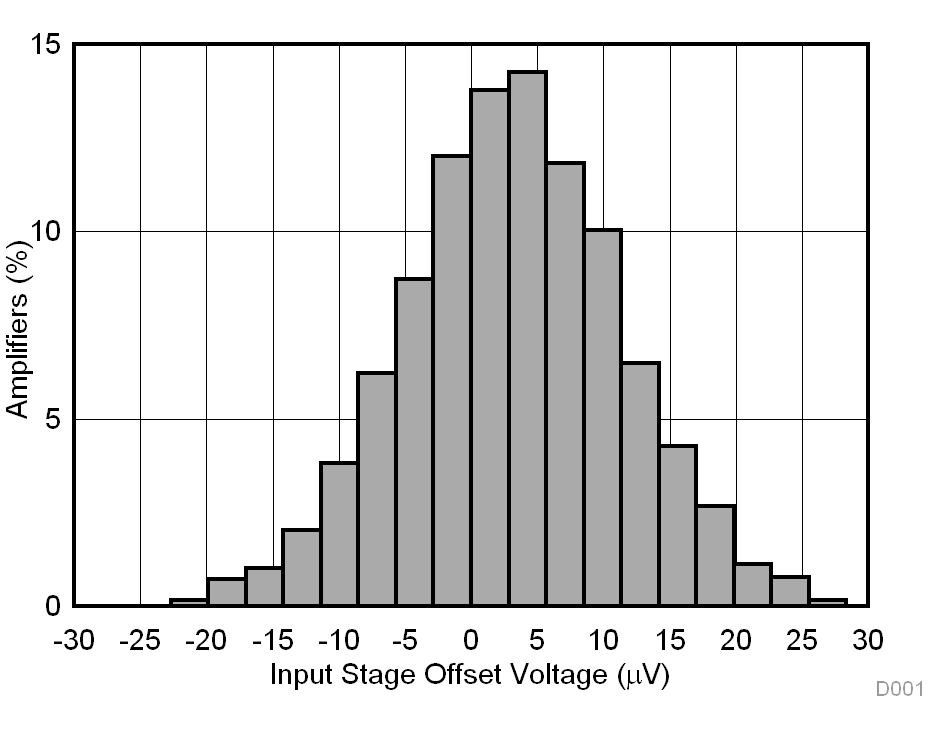N = 2667 Mean = 3.1 µV Std. Dev. = 8.1 µV
Figure 1. Typical Distribution of
Input Stage Offset Voltage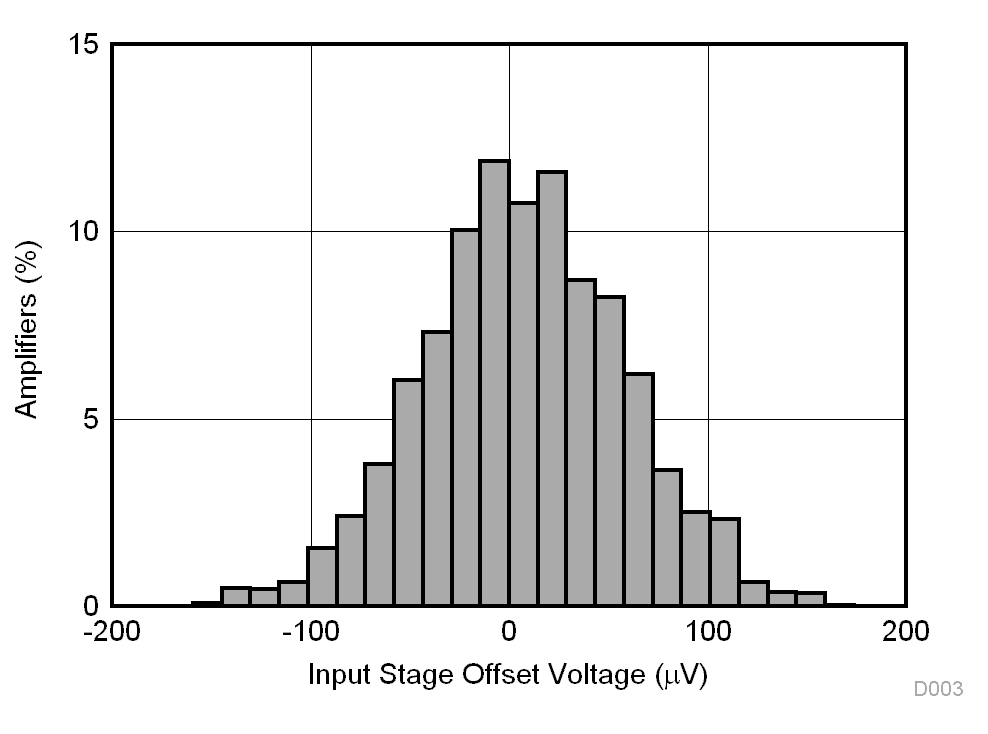N = 2667 Mean = 7.7 µV Std. Dev. = 50.7 µV
Figure 3. Typical Distribution of
Output Stage Offset Voltage81 units
Figure 5. Input Stage Offset Voltage vs Temperature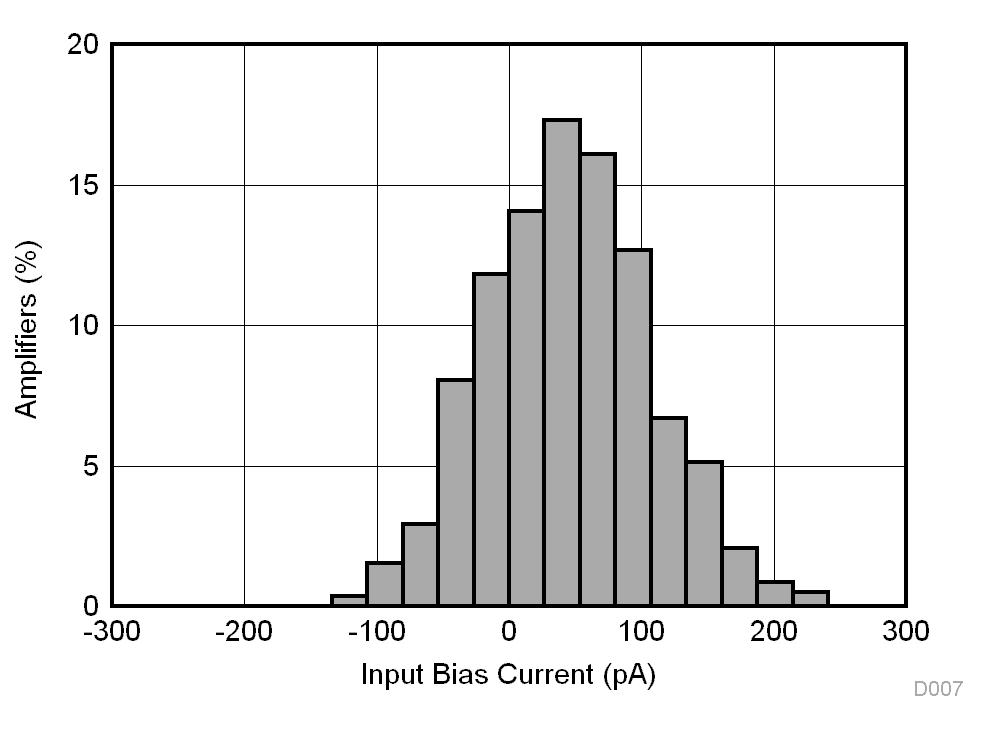N = 292 Mean = 45 pA Std. Dev. = 62 pA TA = 25°C
Figure 7. Typical Distribution of Input Bias Current,
TA = 25°C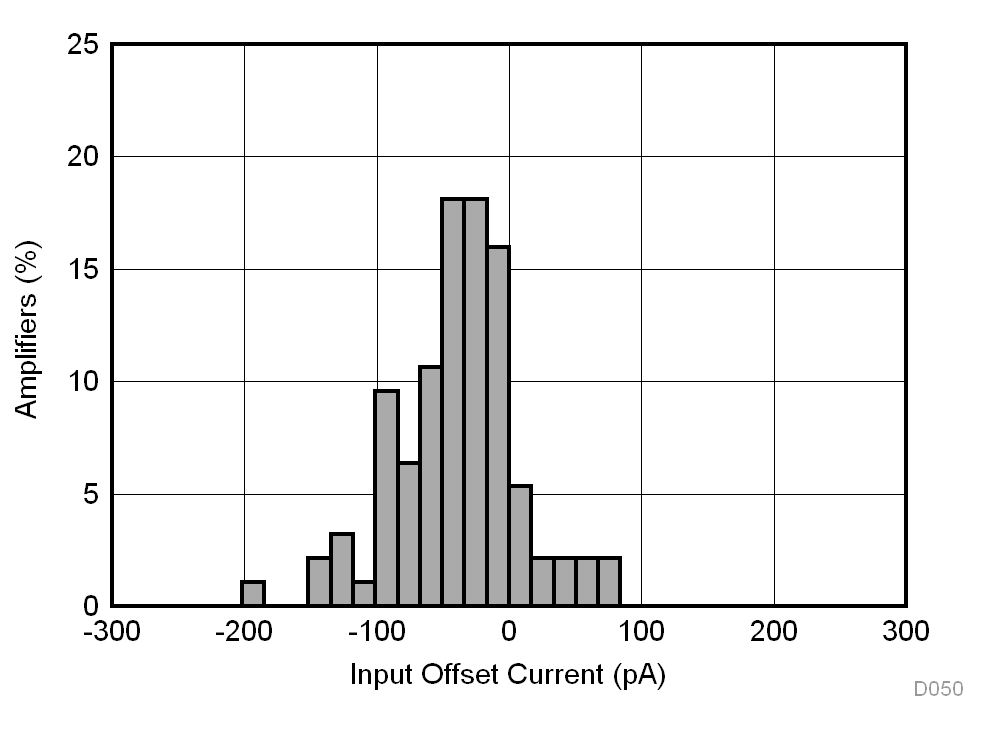N = 94 Mean = –38.82 pA Std. Dev. = 47.24 pA
Figure 9. Typical Distribution of Input Offset Current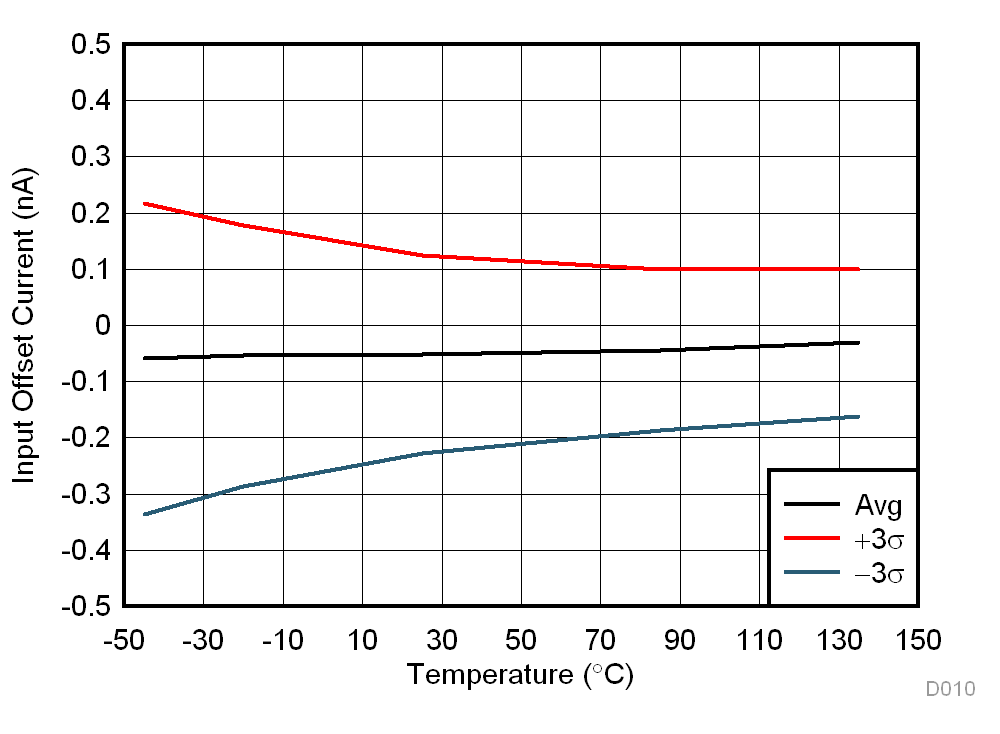N = 294 G = 1
Figure 11. Input Offset Current vs Temperature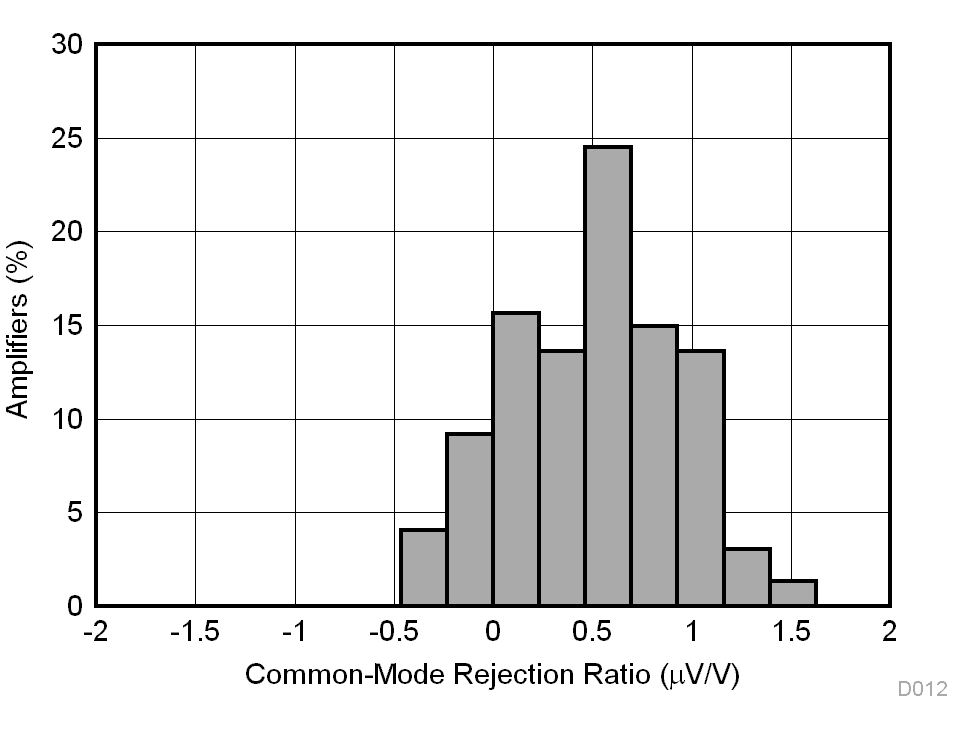N = 294 Mean = 0.51 µV/V Std. Dev. = 0.42 µV/V G = 10
Figure 13. Typical CMRR Distribution, G = 10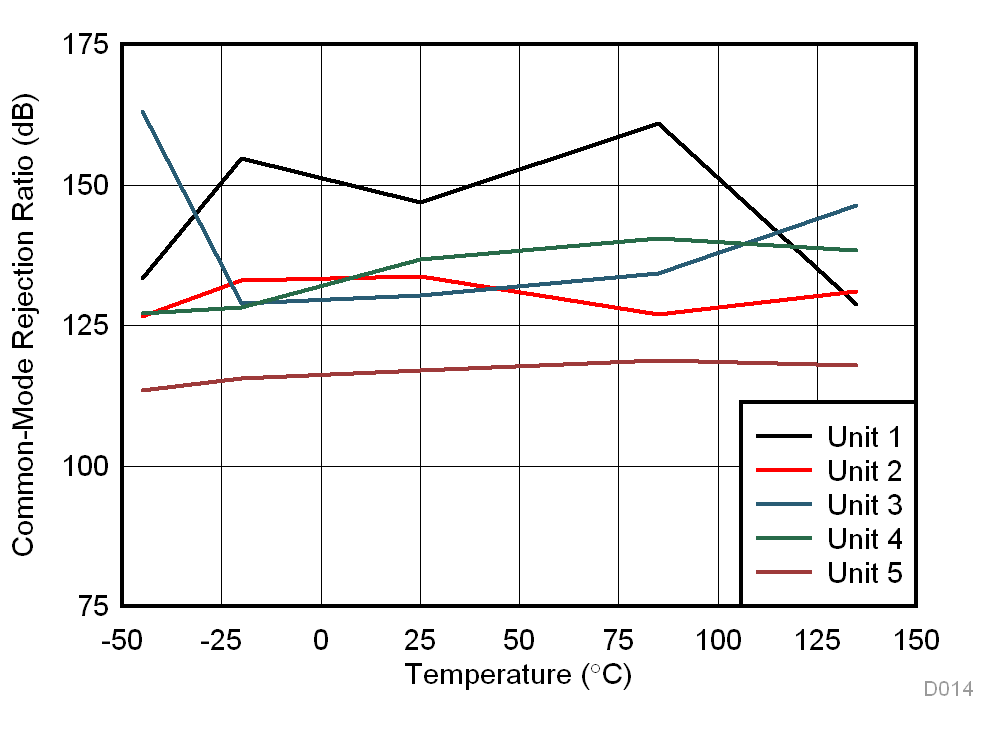N = 5 G = 10
Figure 15. CMRR vs Temperature, G = 10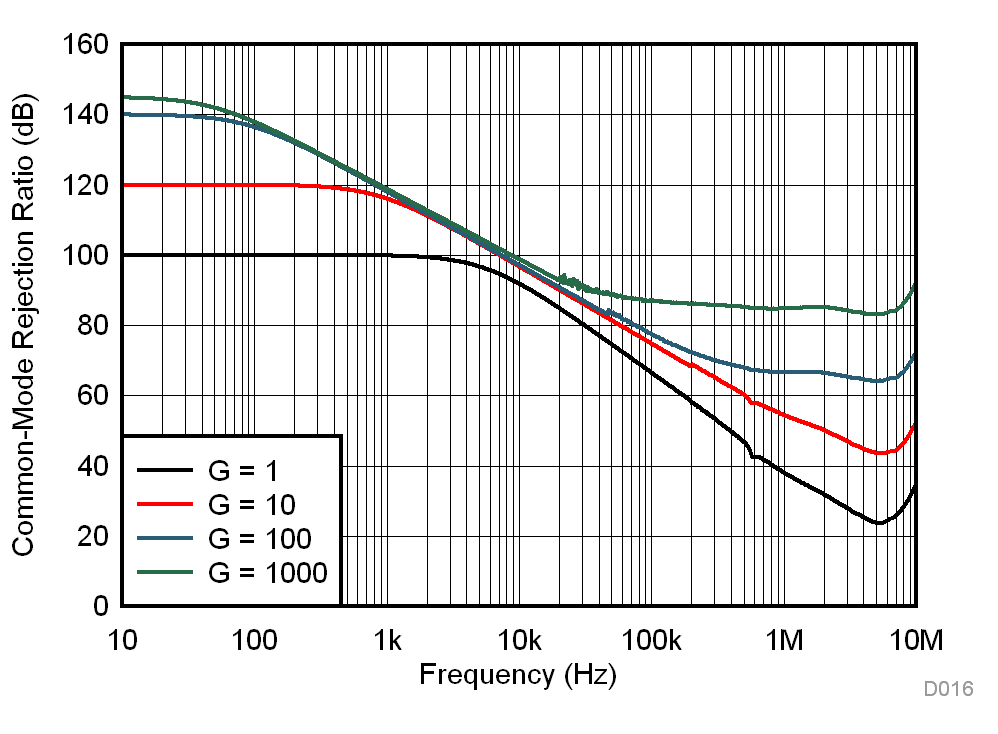Figure 17. CMRR vs Frequency (RTI)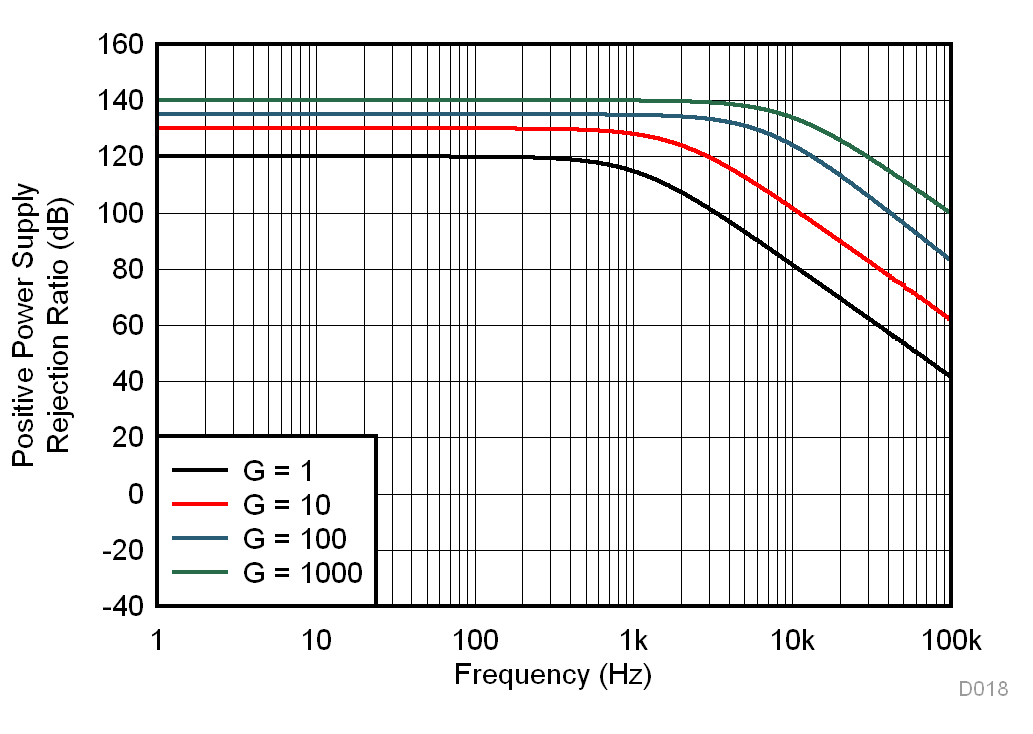Figure 19. Positive PSRR vs Frequency (RTI)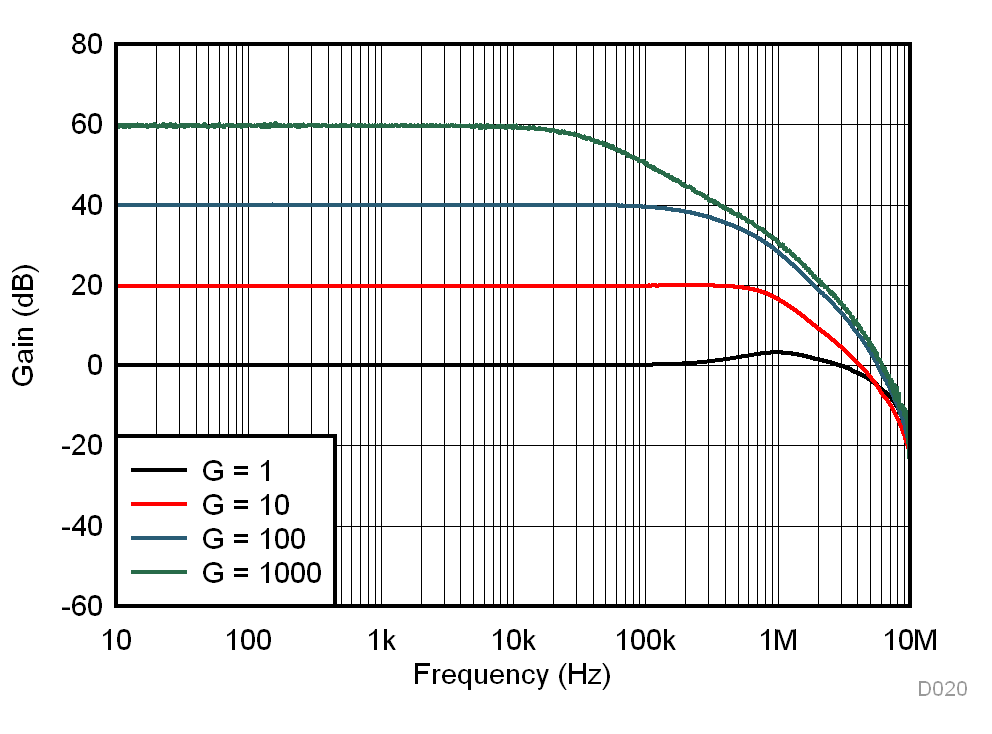Figure 21. Gain vs Frequency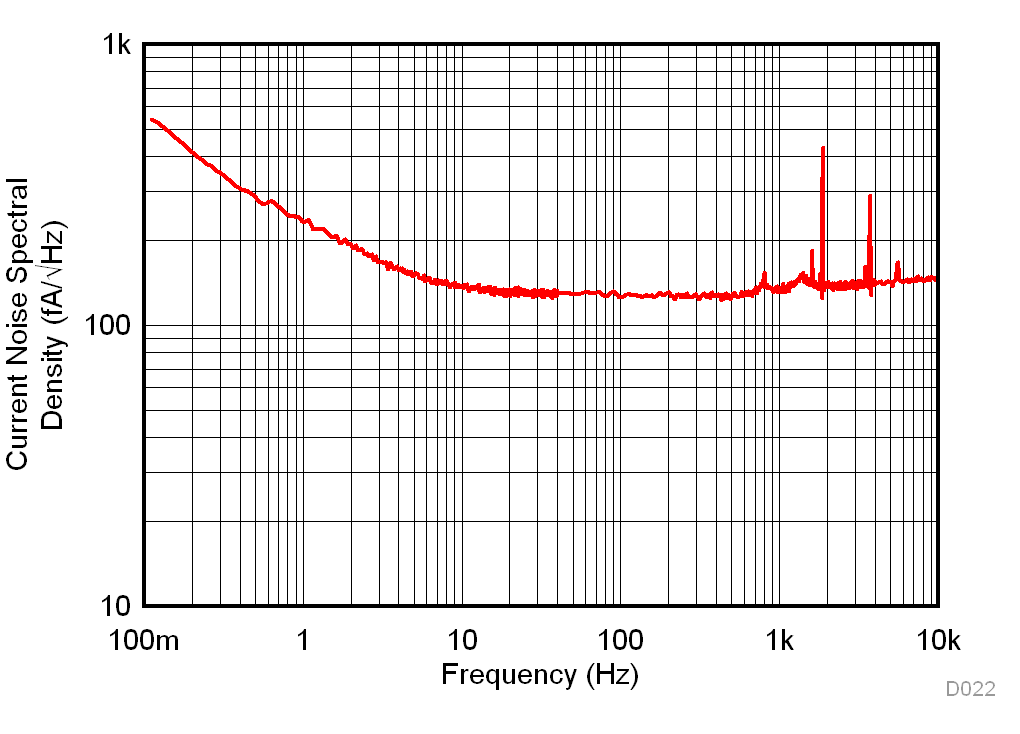Figure 23. Current Noise Spectral Density vs Frequency (RTI)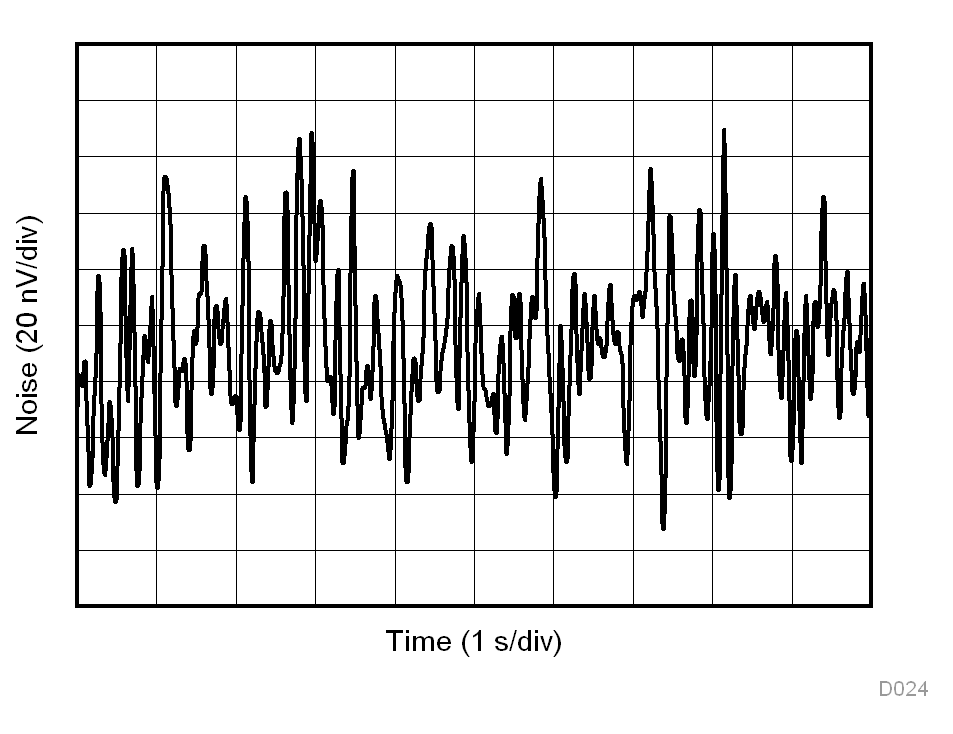G = 1000
Figure 25. 0.1-Hz to 10-Hz RTI Voltage Noise, G = 1000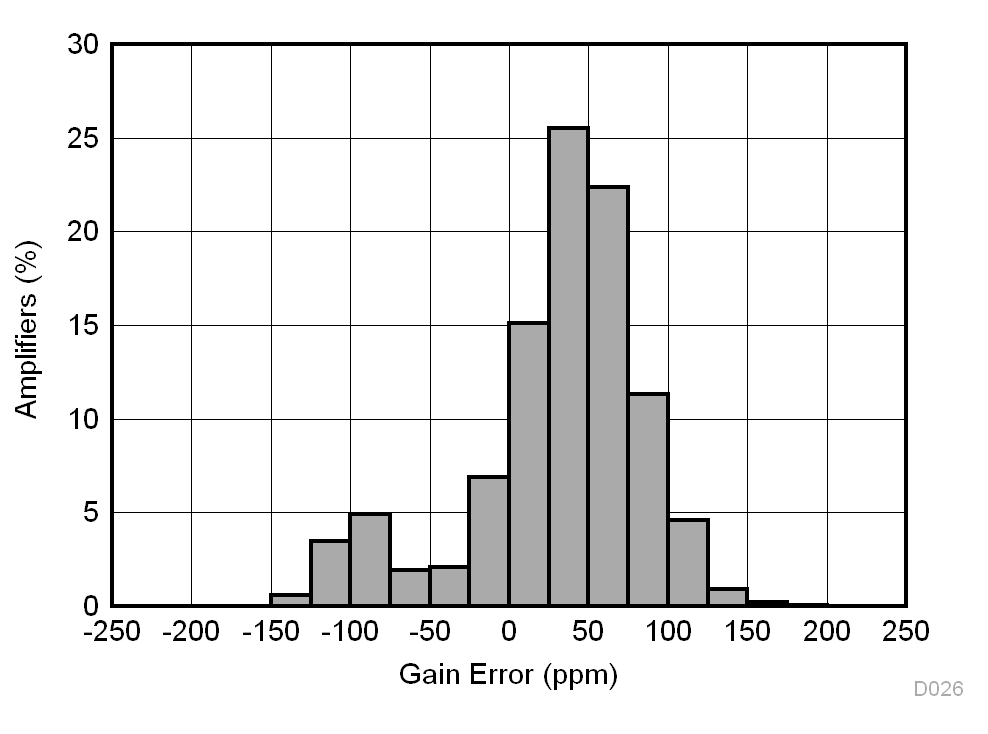N = 5412 Mean = 30 ppm Std. Dev. = 55 ppm G = 1
Figure 27. Typical Distribution of Gain Error, G = 1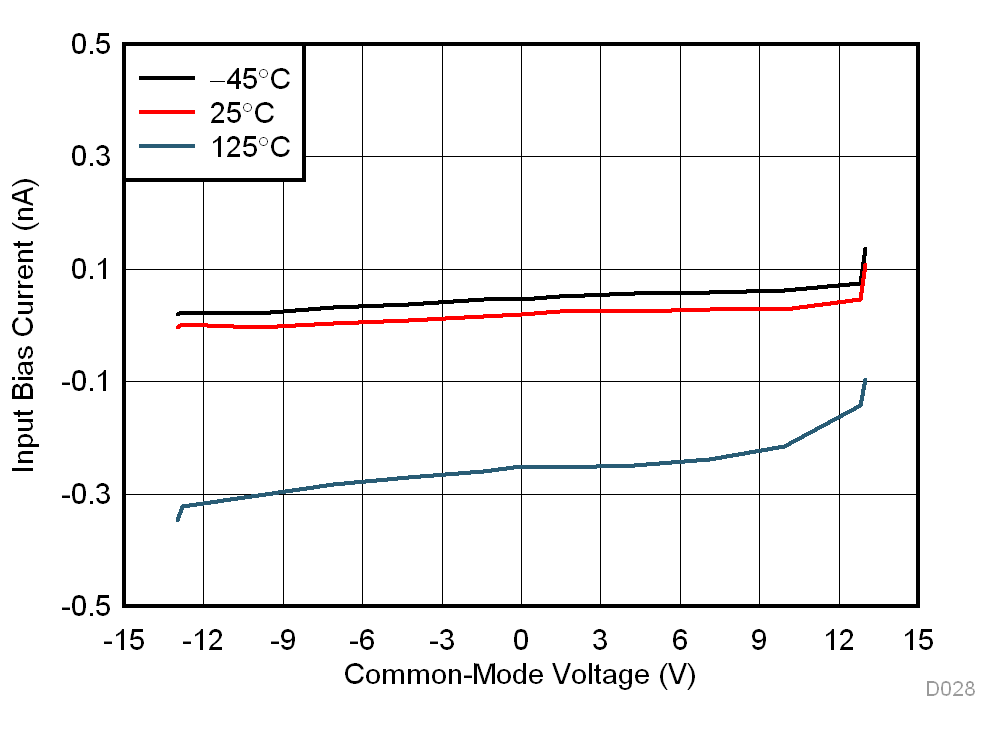VS = ±15 V
Figure 29. Input Bias Current vs Common-Mode Voltage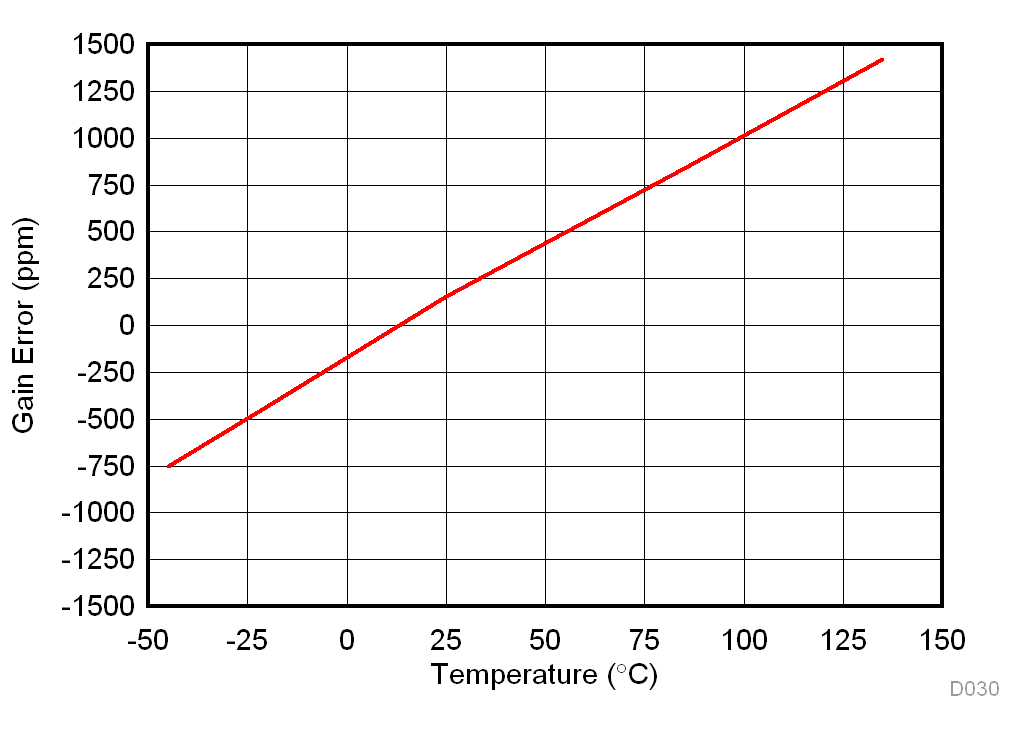Average of 294 units G = 10
Figure 31. Gain Error vs Temperature, G = 10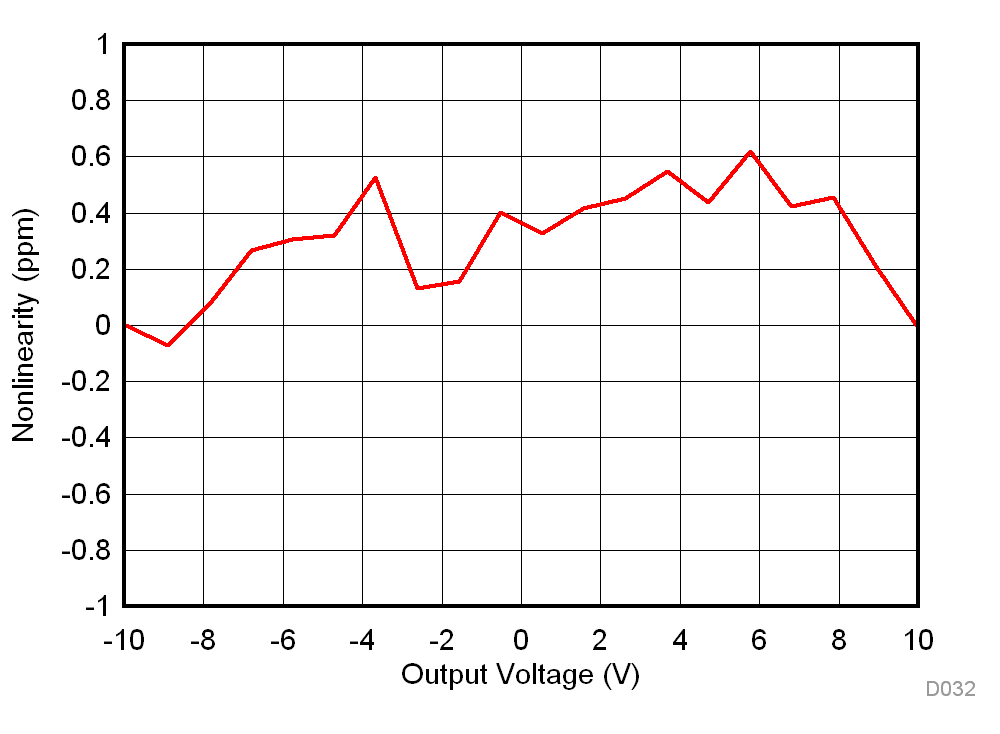G = 1
Figure 33. Gain Nonlinearity, G = 1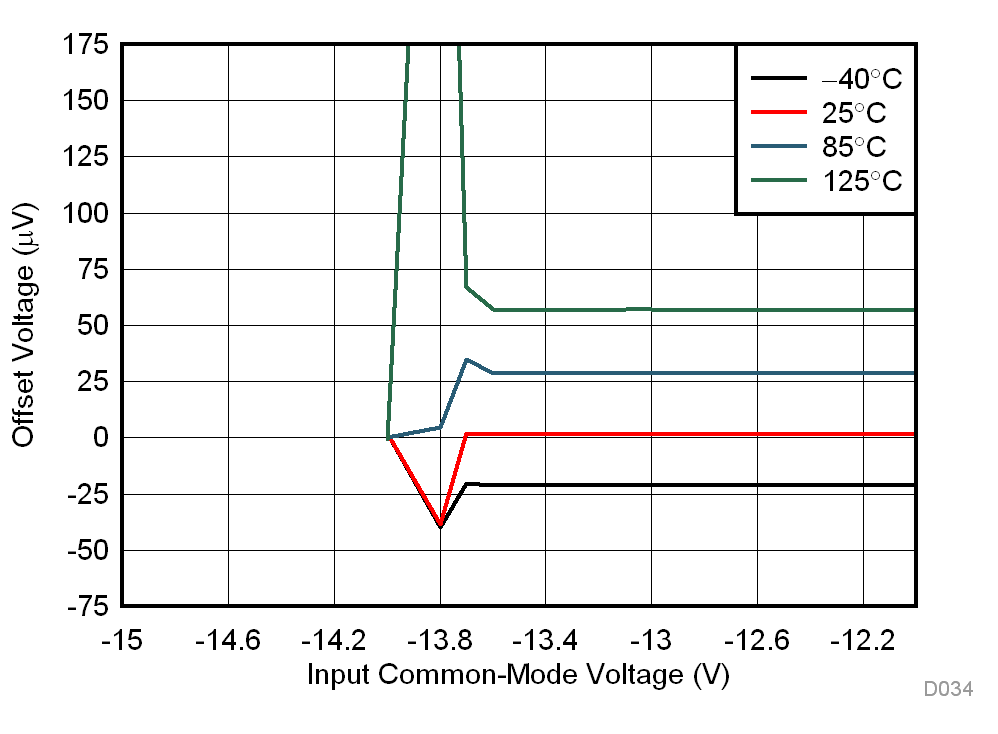Figure 35. Offset Voltage vs Negative Common-Mode Voltage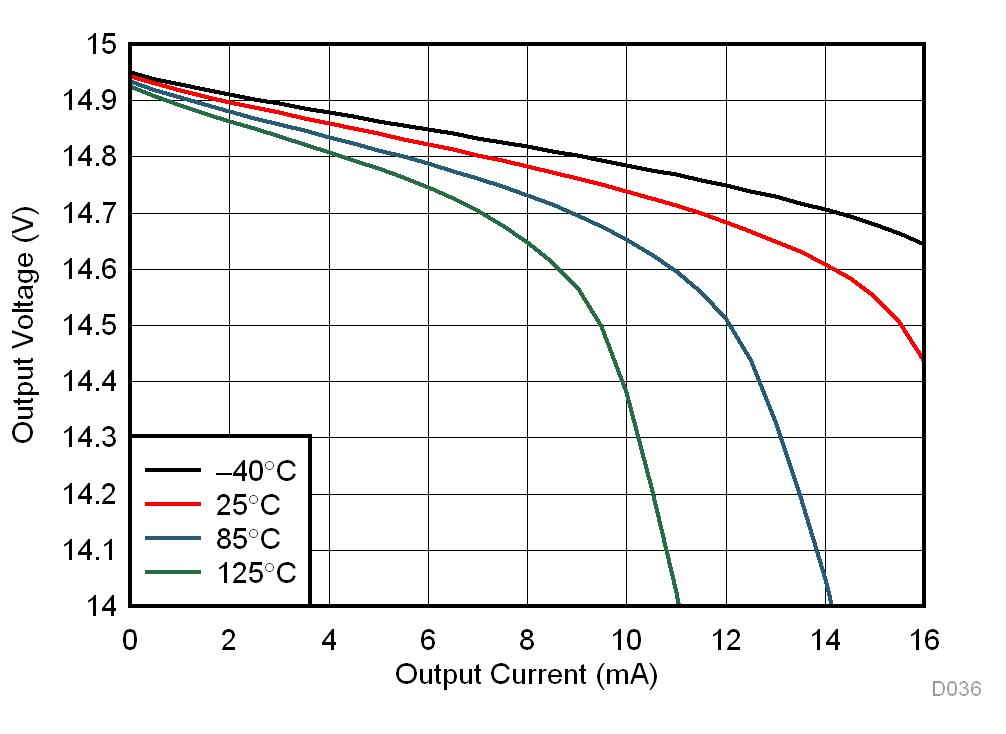Figure 37. Positive Output Voltage Swing vs Output Current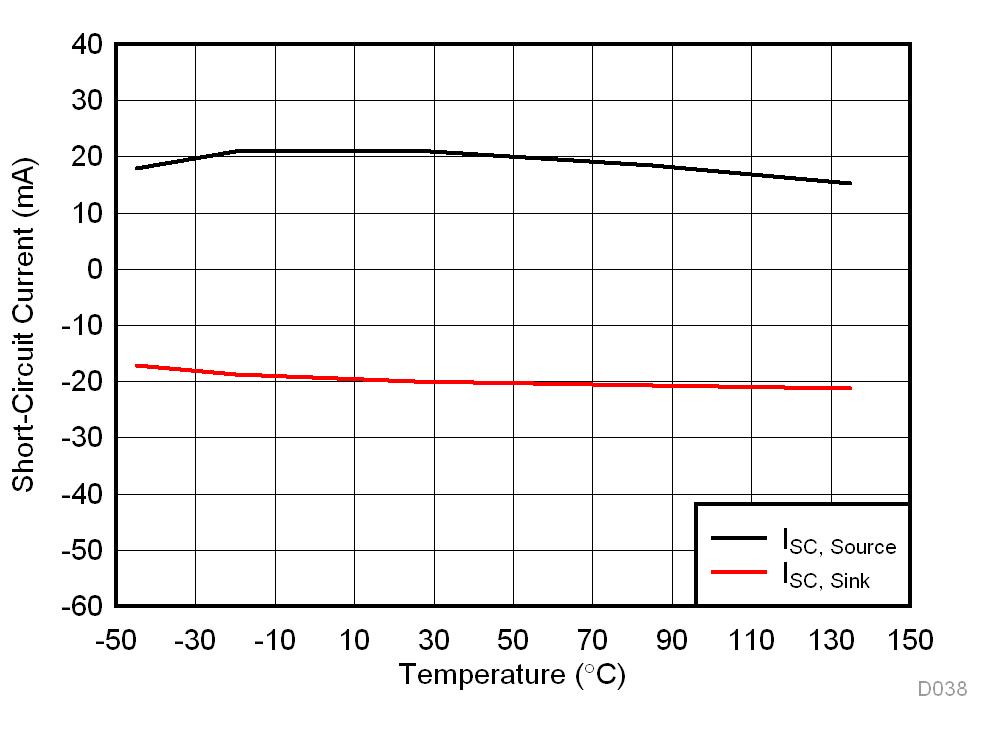Figure 39. Short-Circuit Current vs Temperature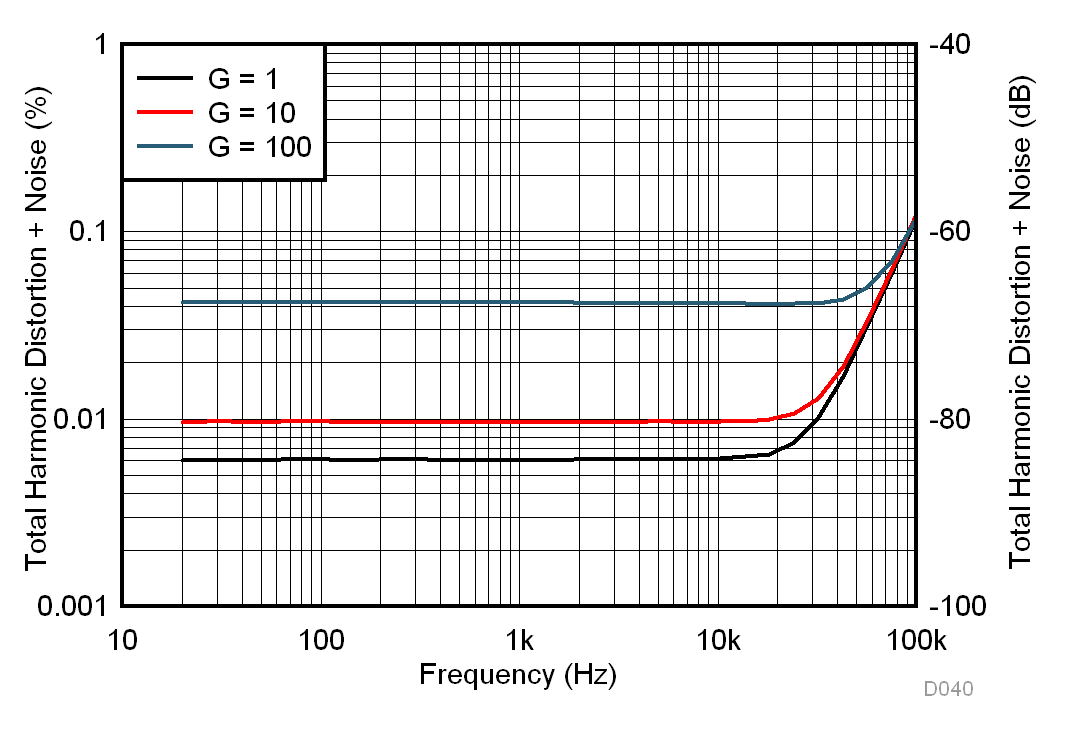500-kHz measurement bandwidth 1-VRMS output voltage 100-kΩ load
Figure 41. THD+N vs Frequency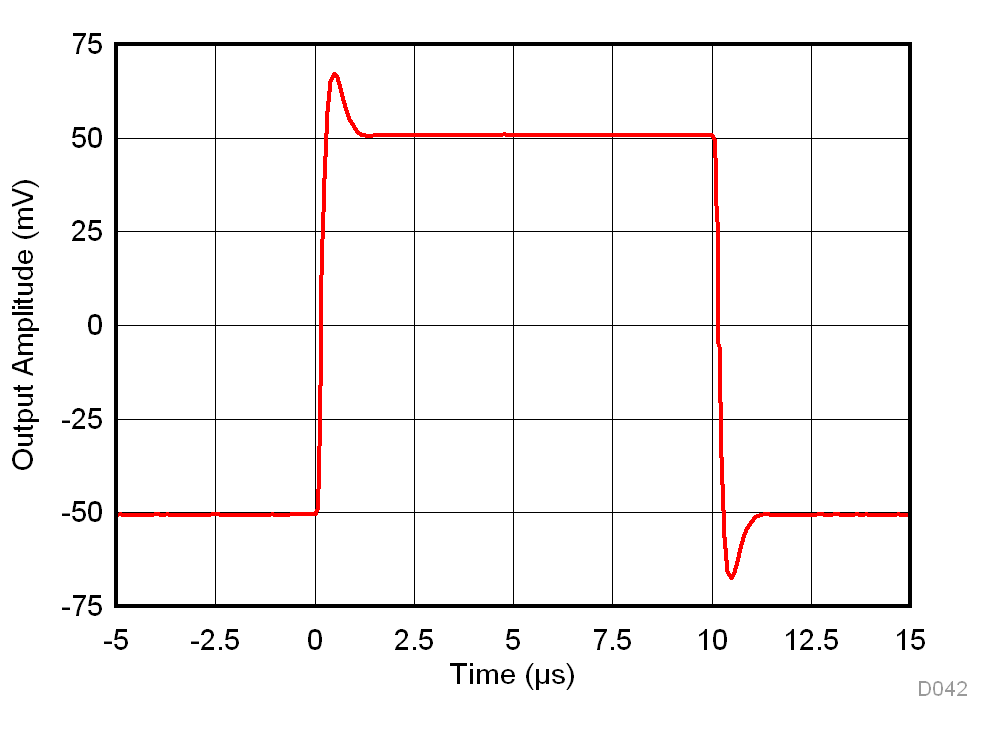G = 1 RL = 10 kΩ CL = 100 pF
Figure 43. Small-Signal Response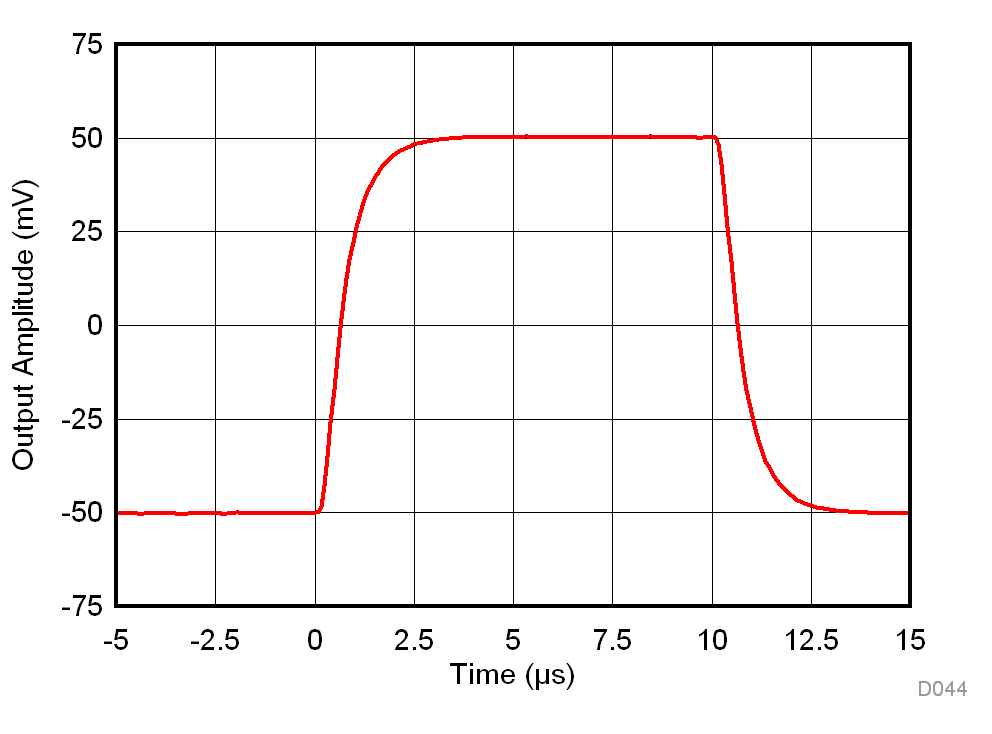G = 100 RL = 10 kΩ CL = 100 pF
Figure 45. Small-Signal Response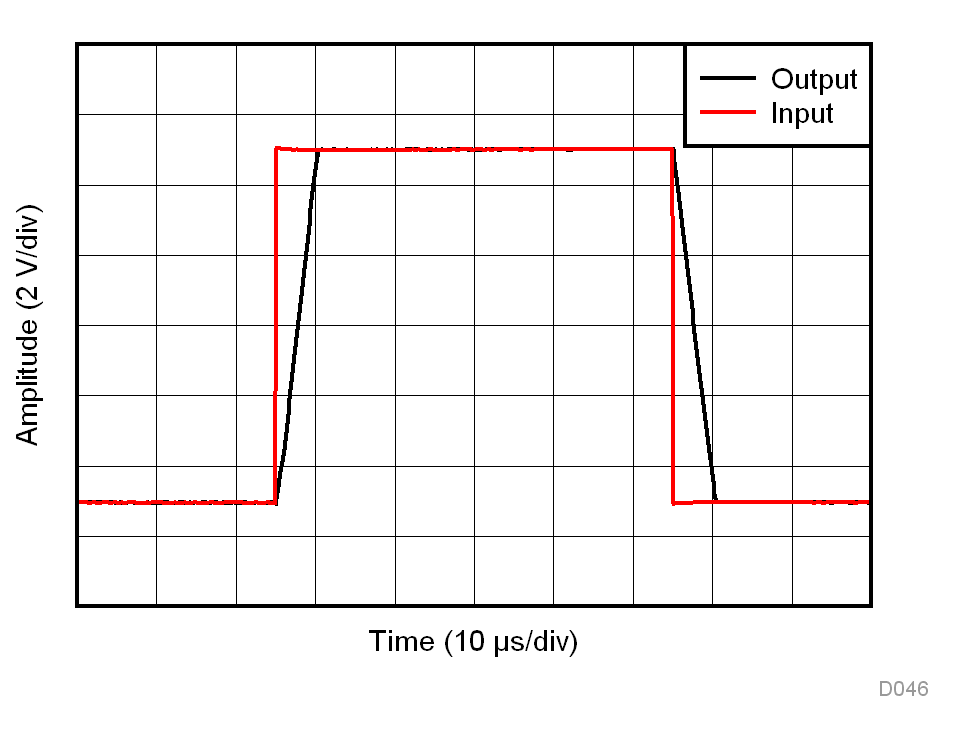Figure 47. Large-Signal Step Response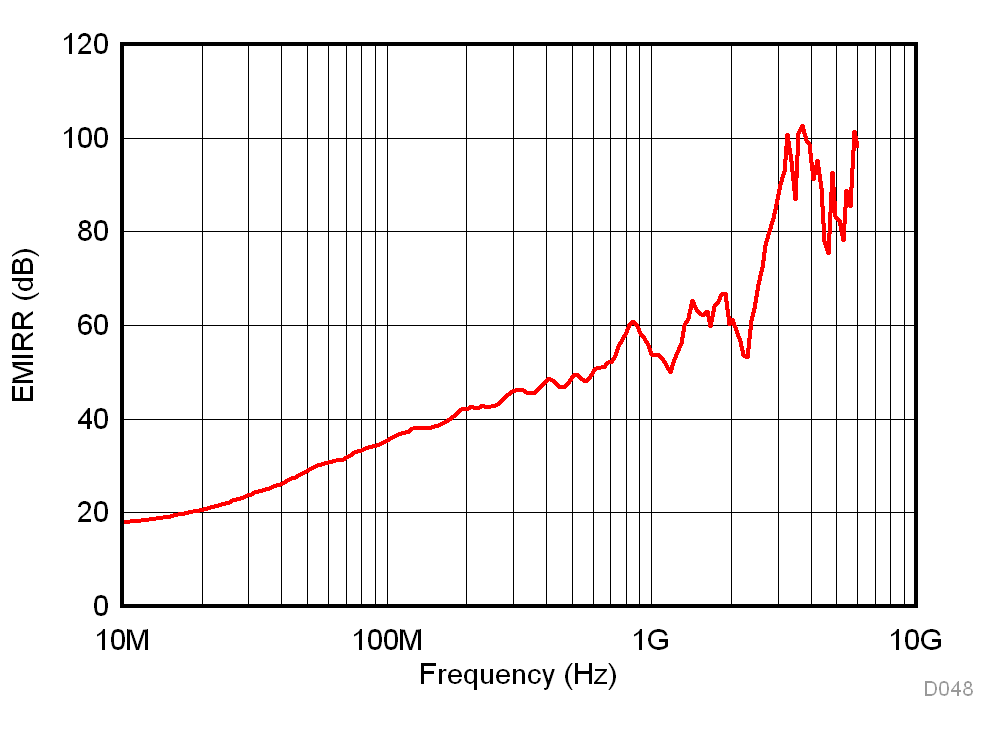Figure 49. Differential-Mode EMI Rejection RatioVS = 5 V G = 1
Figure 51. Input Common-Mode Voltage vs Output Voltage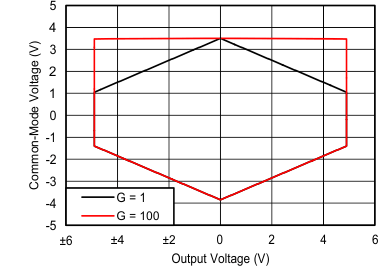VS = ±5 V VREF = 0 V
Figure 53. Input Common-Mode Voltage vs Output Voltage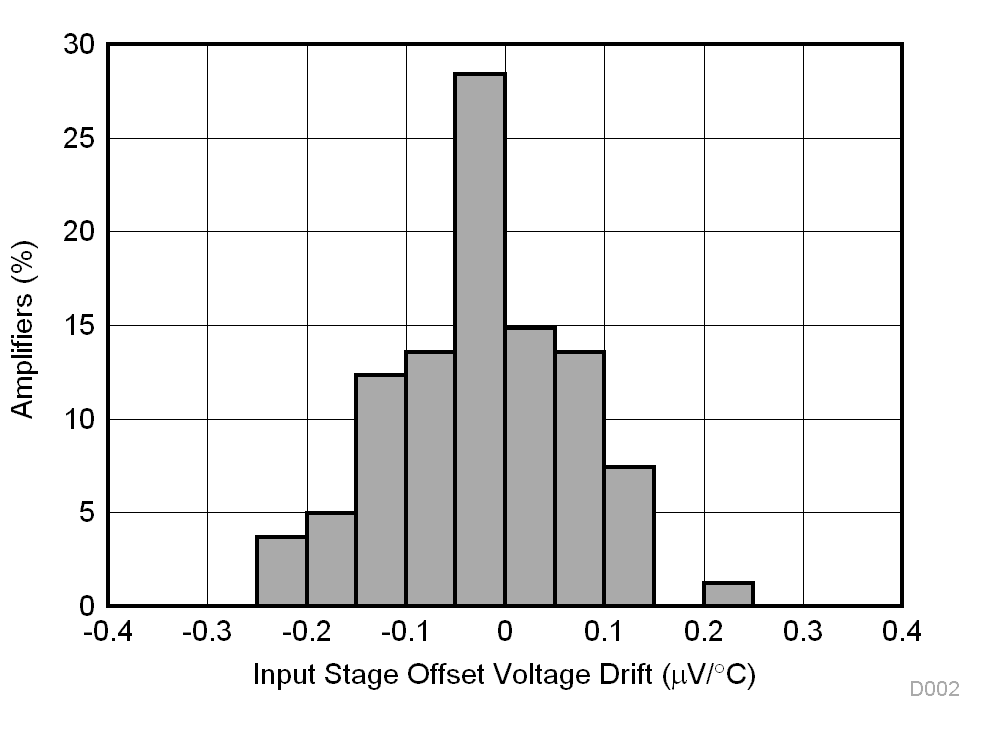N = 81 Mean = -0.03 µV/°C Std. Dev. = 0.09 µV/°C
Figure 2. Typical Distribution of
Input Stage Offset Voltage Drift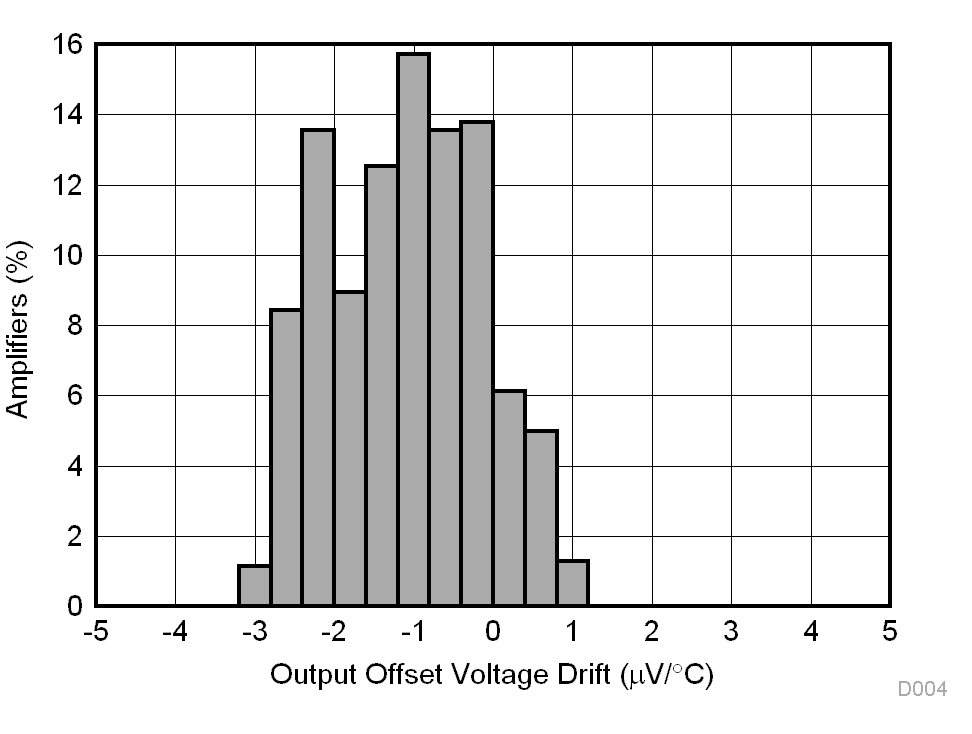N = 81 Mean = –1.09 µV/°C Std. Dev. = 0.94 µV/°C
Figure 4. Typical Distribution of
Output Stage Offset Voltage Drift81 units
Figure 6. Output Stage Offset Voltage vs Temperature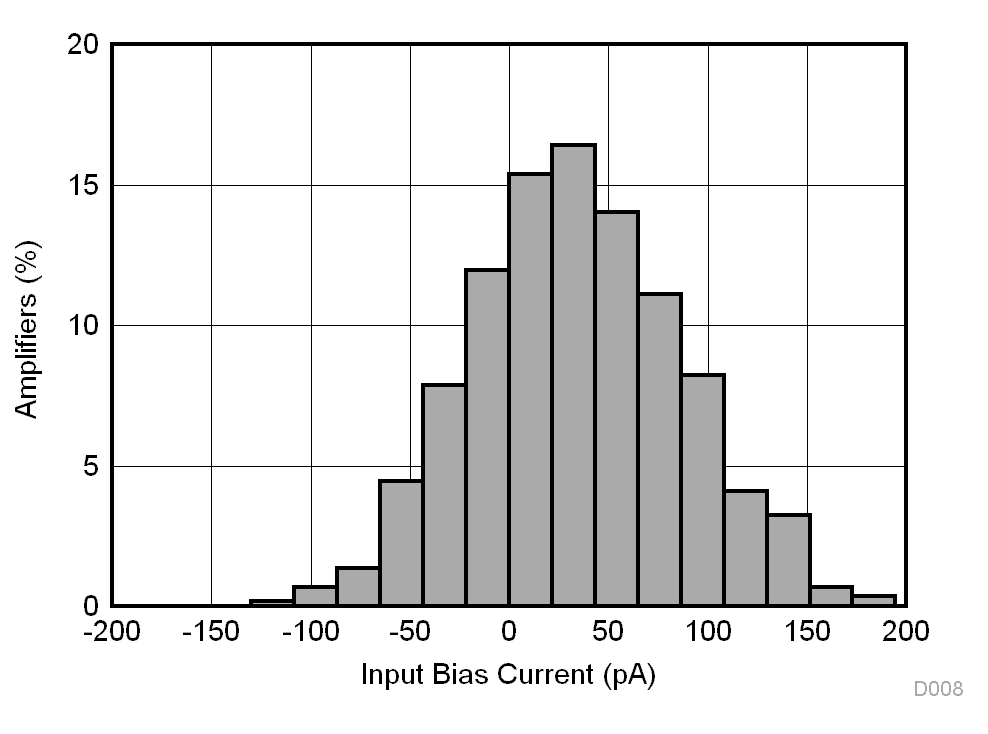N = 292 Mean = 34 pA Std. Dev. = 52 pA TA = 90°C
Figure 8. Typical Distribution of Input Bias Current,
TA = 90°C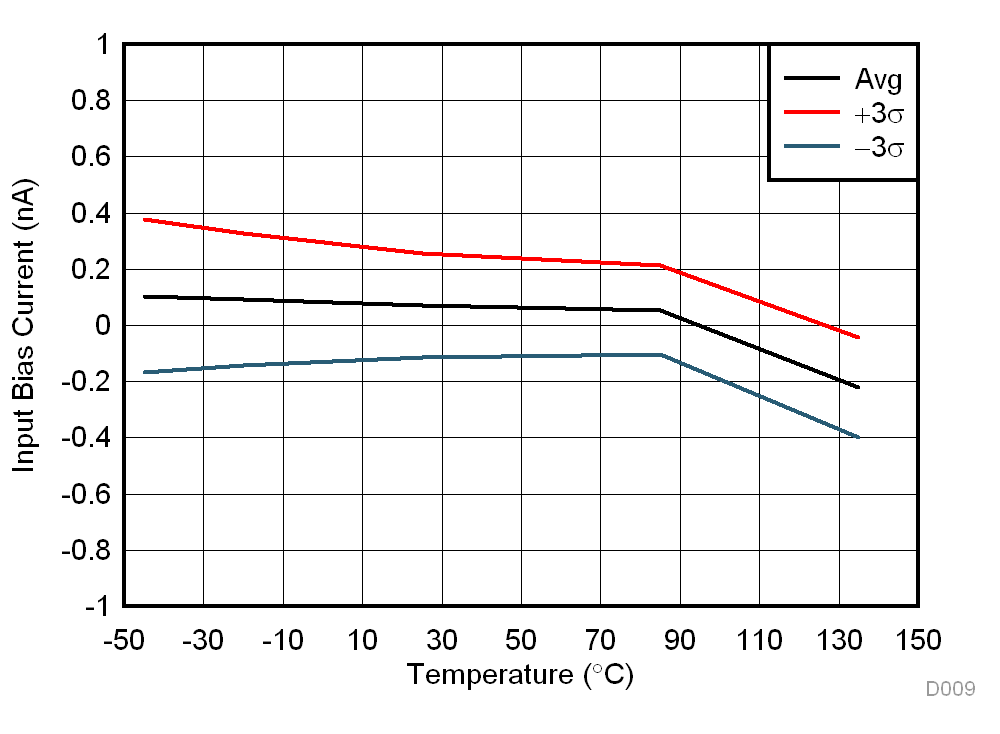N = 294 G = 1
Figure 10. Input Bias Current vs Temperature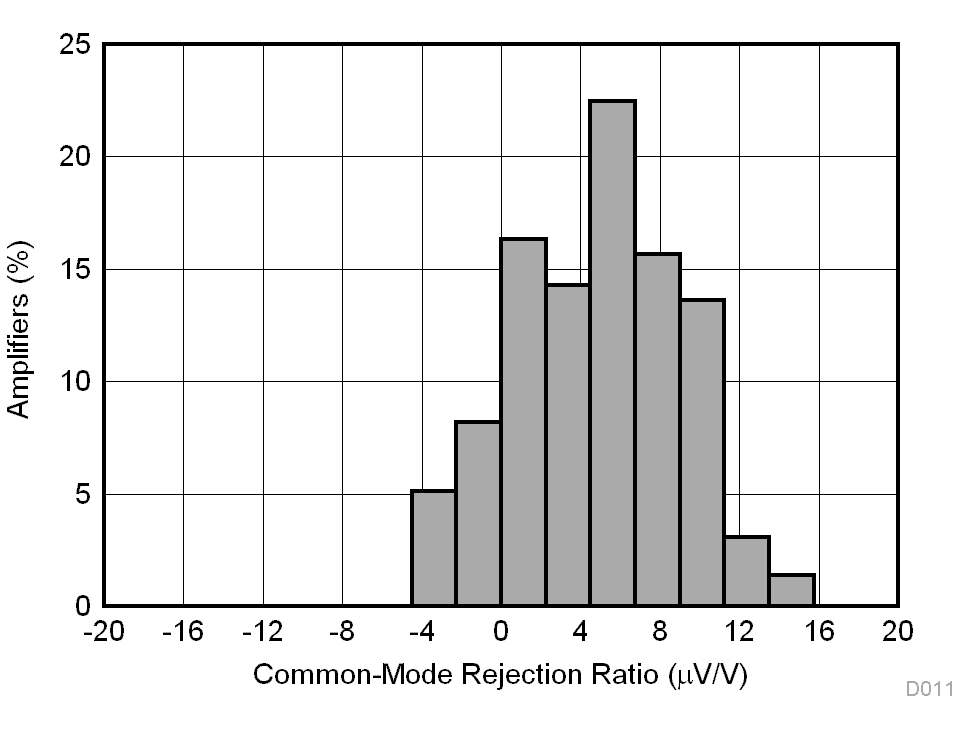N = 294 Mean = 4.87 µV/V Std. Dev. = 4.14 µV/V G = 1
Figure 12. Typical CMRR Distribution, G = 1N = 5 G = 1
Figure 14. CMRR vs Temperature, G = 1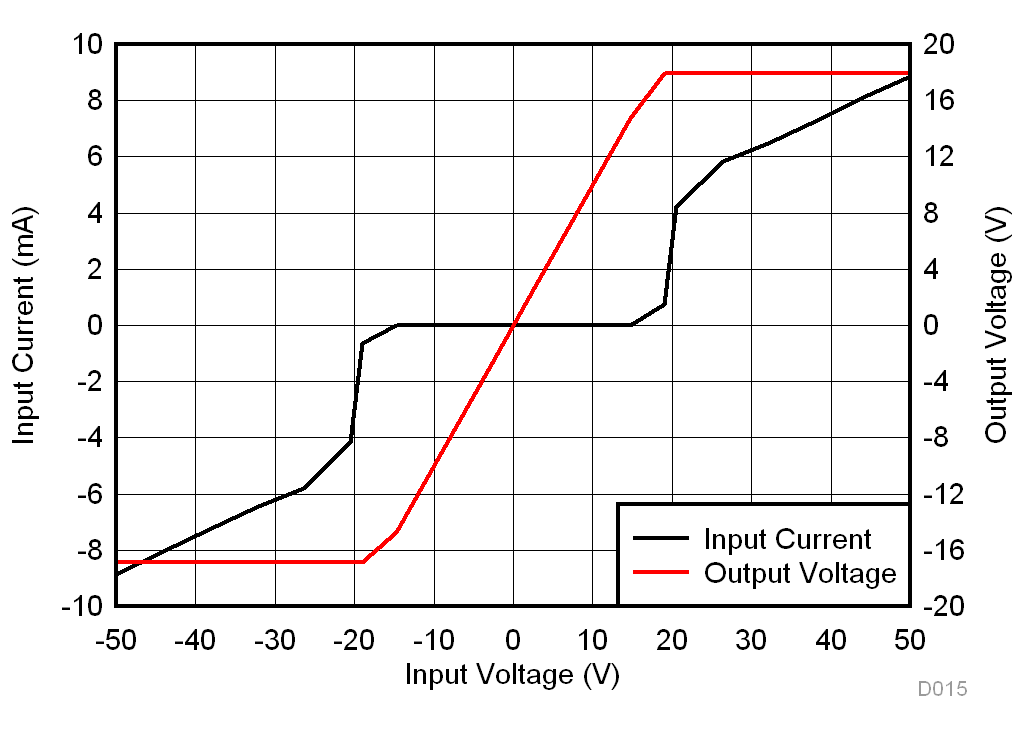VS = ±18 V
Figure 16. Input Current vs Input Overvoltage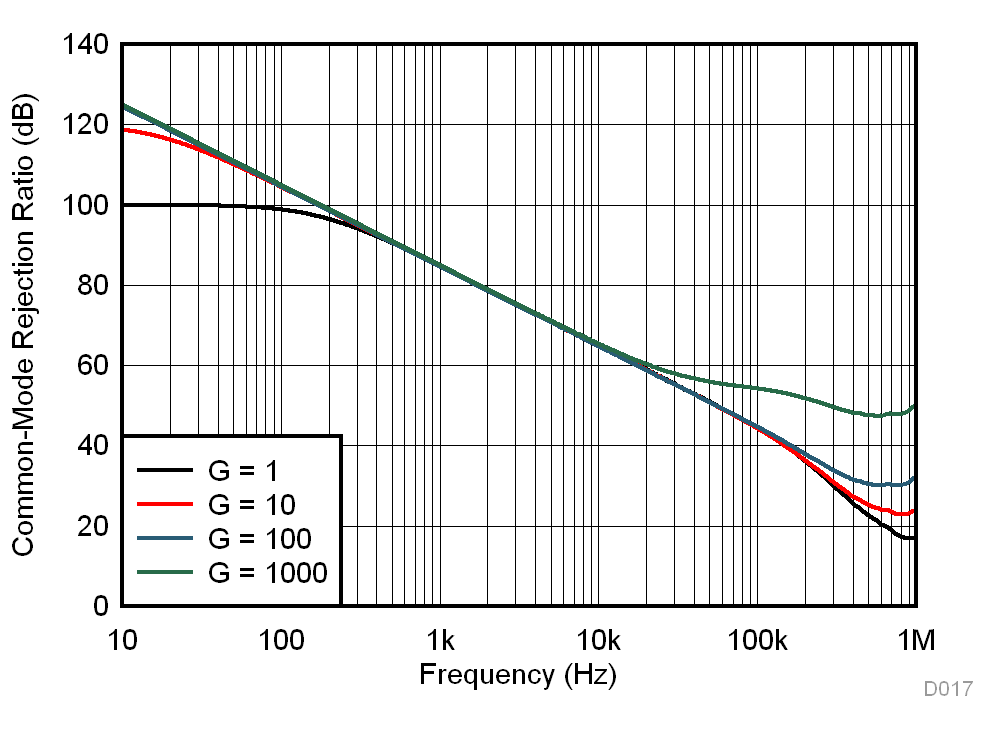Figure 18. CMRR vs Frequency
(RTI, 1-kΩ source imbalance)Figure 20. Negative PSRR vs Frequency (RTI)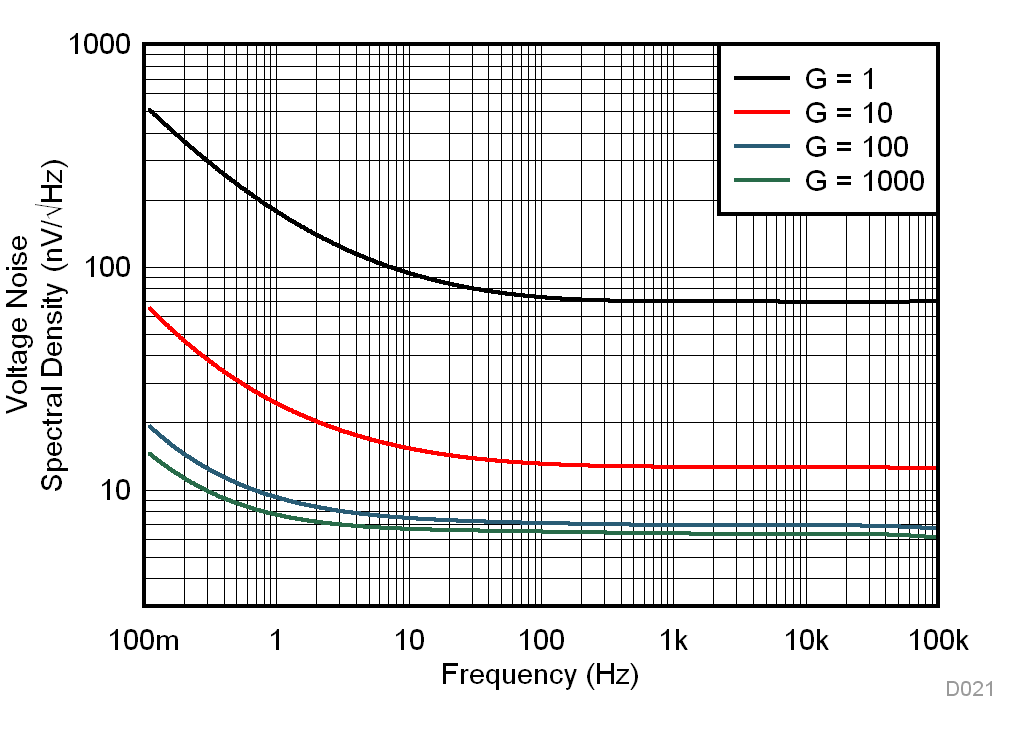Figure 22. Voltage Noise Spectral Density vs Frequency (RTI)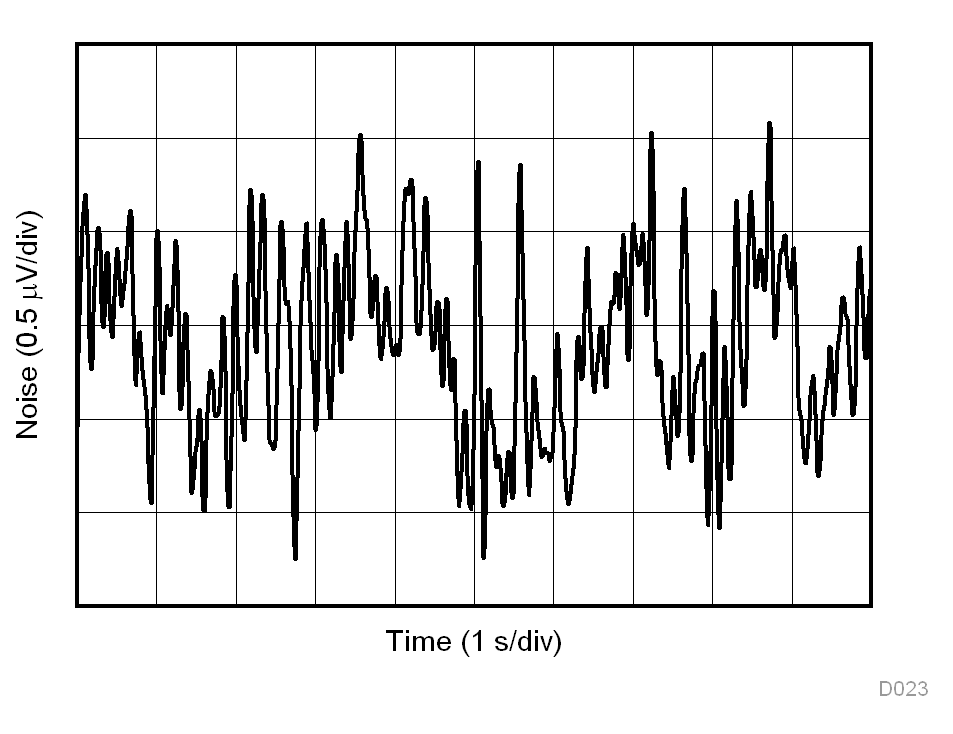G = 1
Figure 24. 0.1-Hz to 10-Hz RTI Voltage Noise, G = 1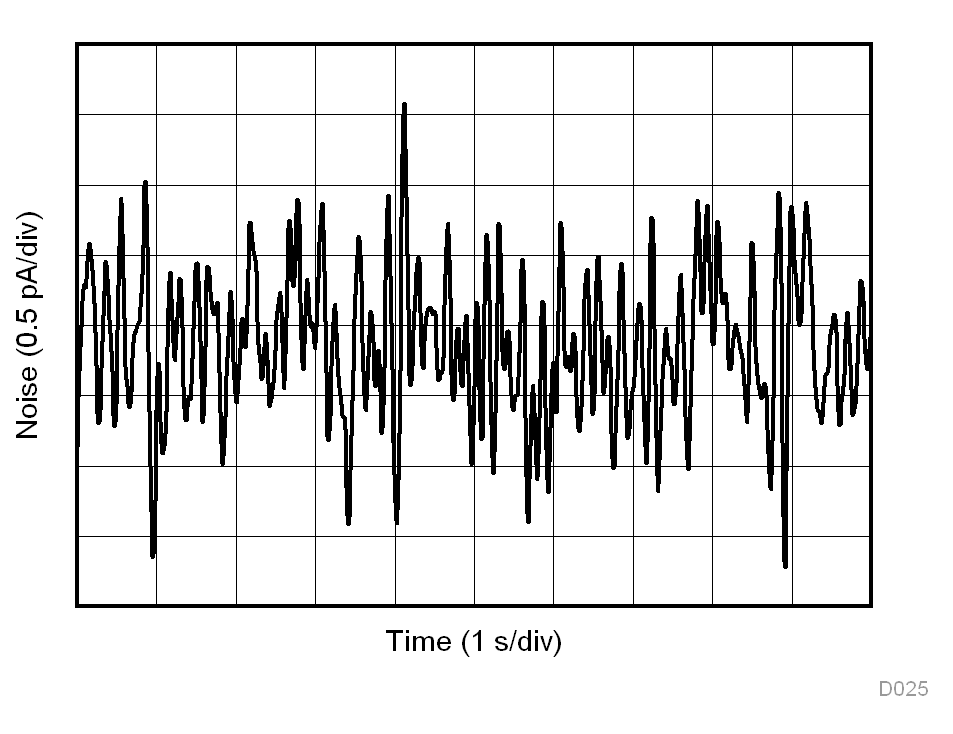G = 1
Figure 26. 0.1-Hz to 10-Hz RTI Current Noise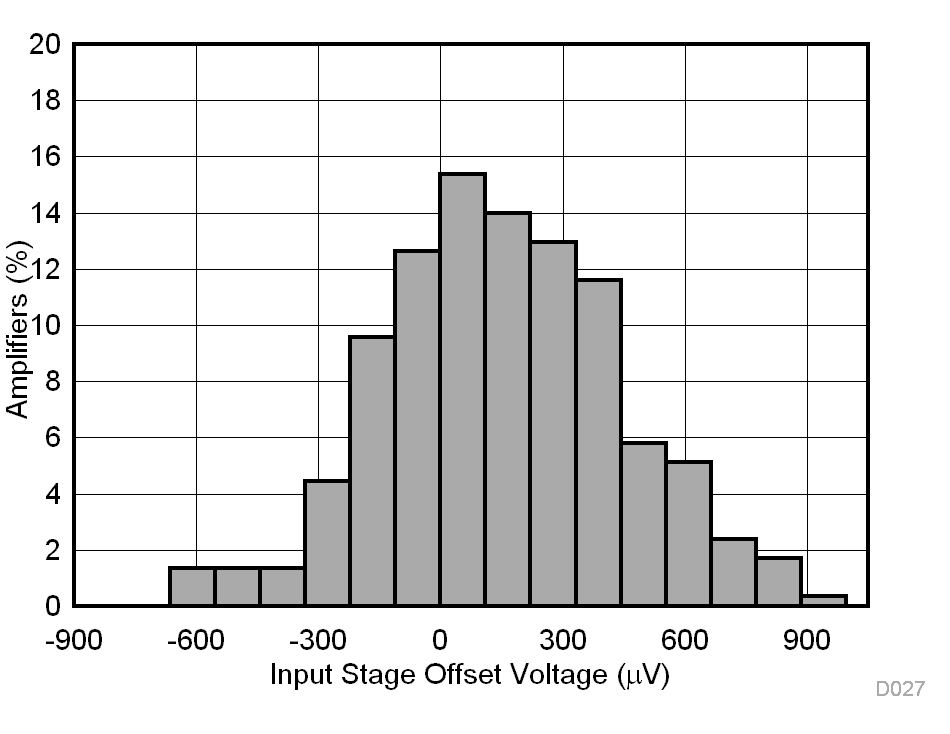N = 293 Mean = 152 ppm Std. Dev. = 291 ppm G = 10
Figure 28. Typical Distribution of Gain Error, G = 10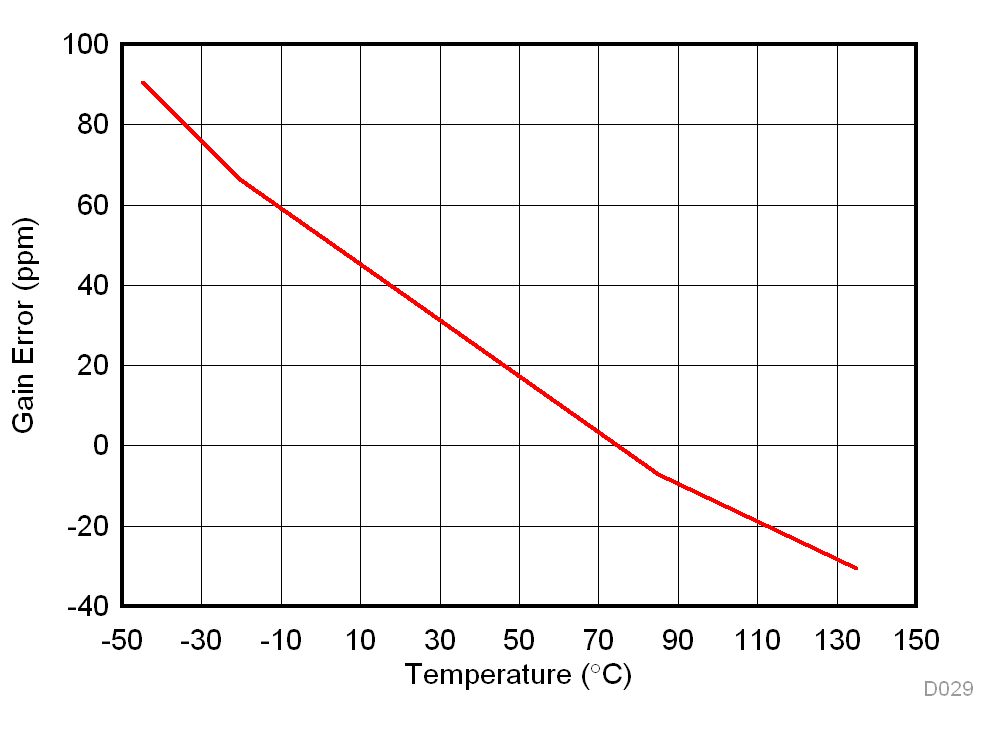Average of 294 units G = 1
Figure 30. Gain Error vs Temperature, G = 1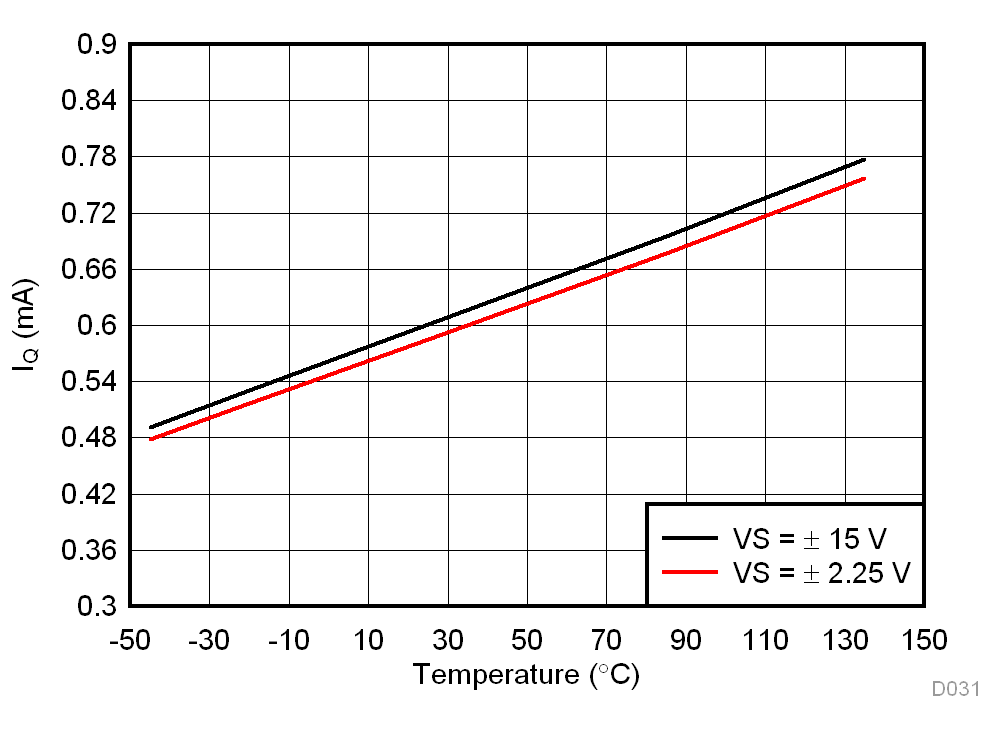Figure 32. Supply Current vs Temperature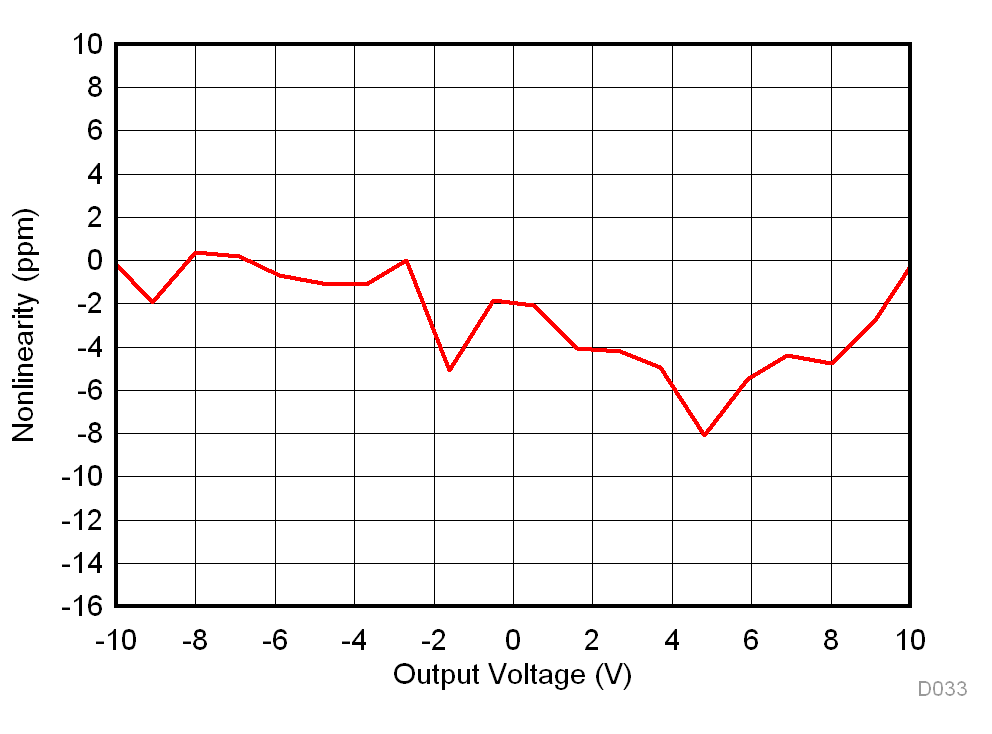G = 10
Figure 34. Gain Nonlinearity, G = 10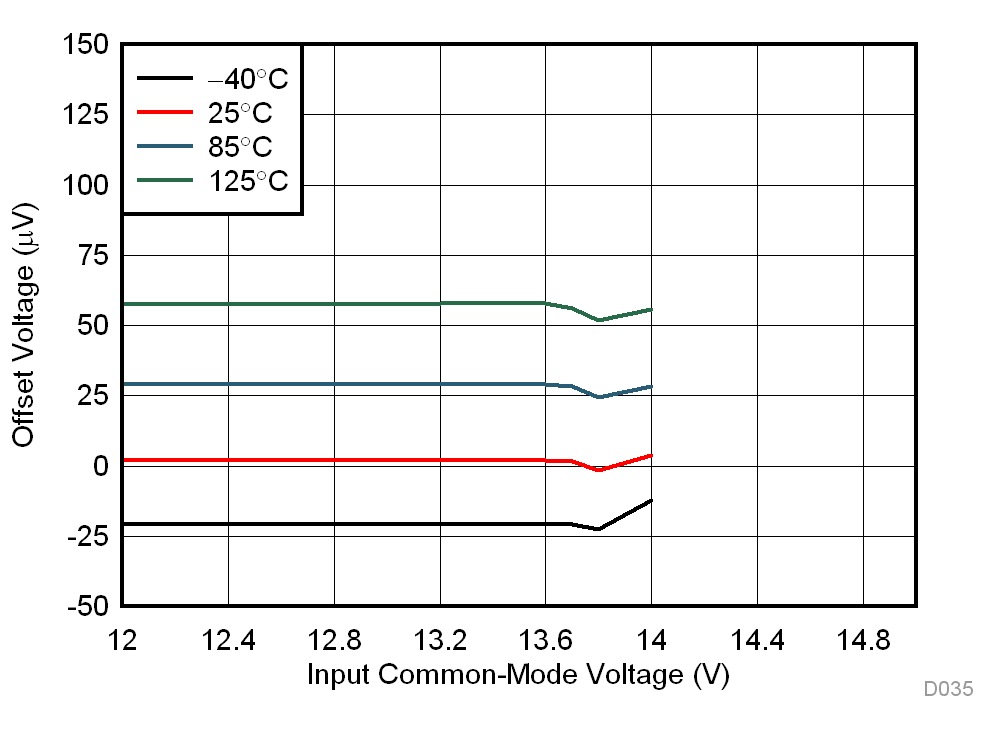Figure 36. Offset Voltage vs Positive Common-Mode Voltage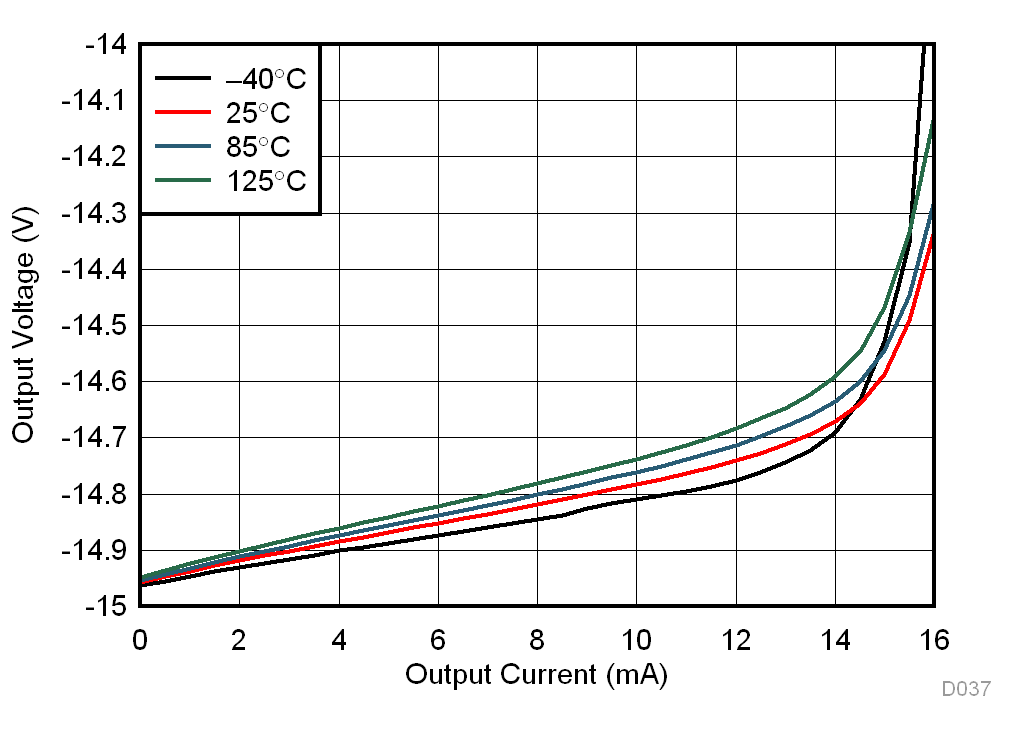Figure 38. Negative Output Voltage Swing vs Output Current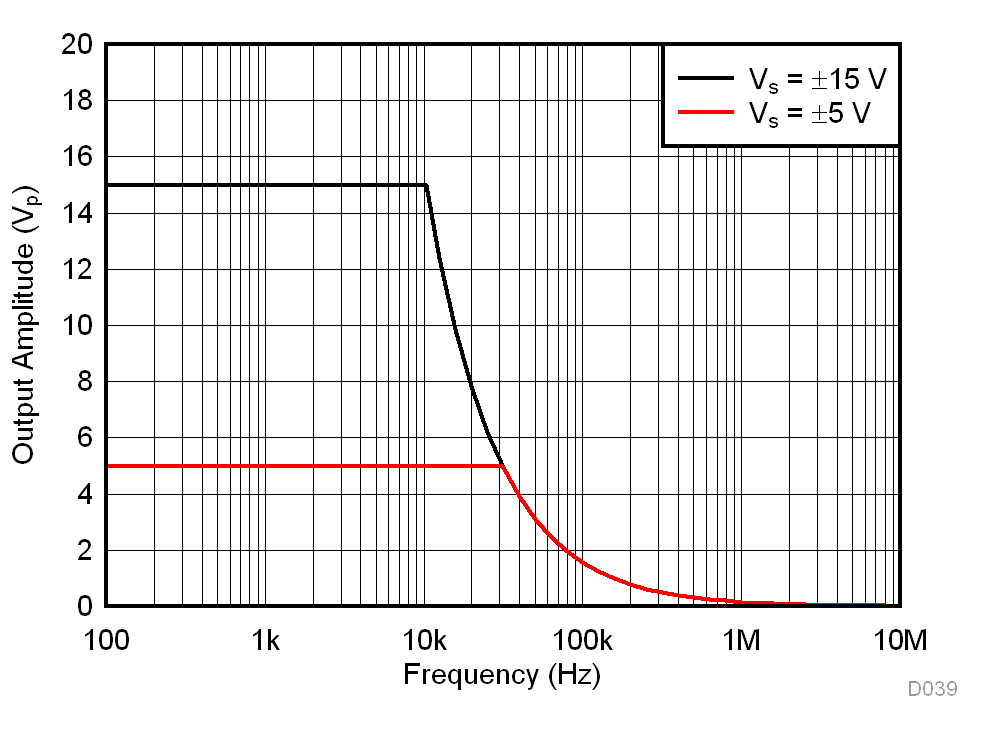Figure 40. Large-Signal Frequency ResponseFigure 42. Overshoot vs Capacitive Loads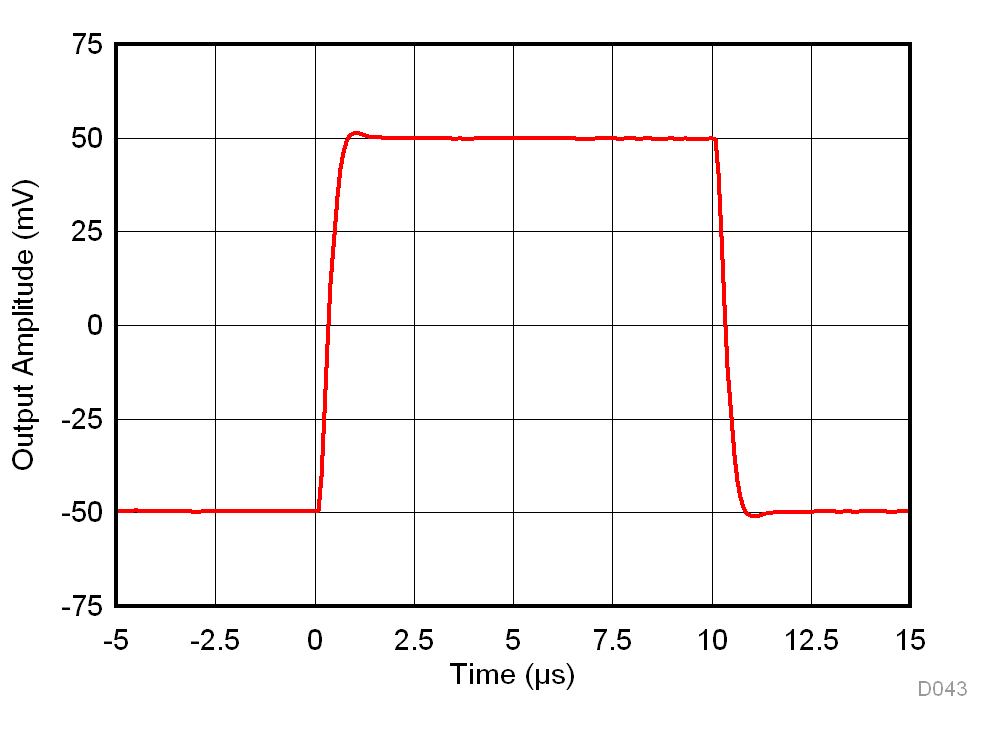G = 10 RL = 10 kΩ CL = 100 pF
Figure 44. Small-Signal Response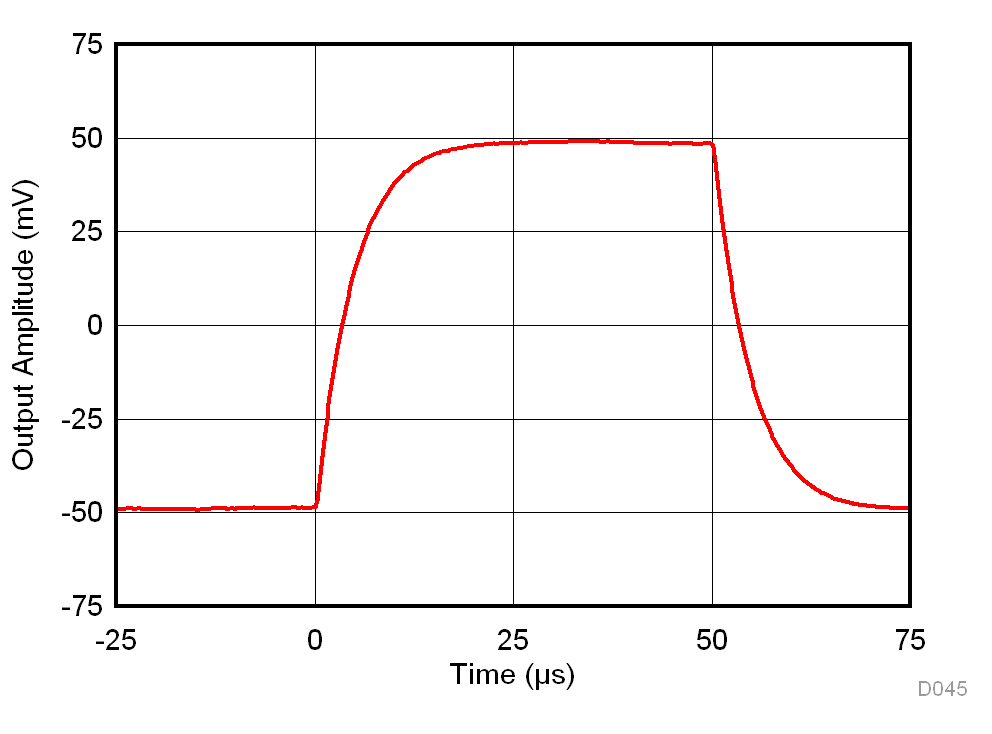G = 1000 RL = 10 kΩ CL = 100 pF
Figure 46. Small-Signal Response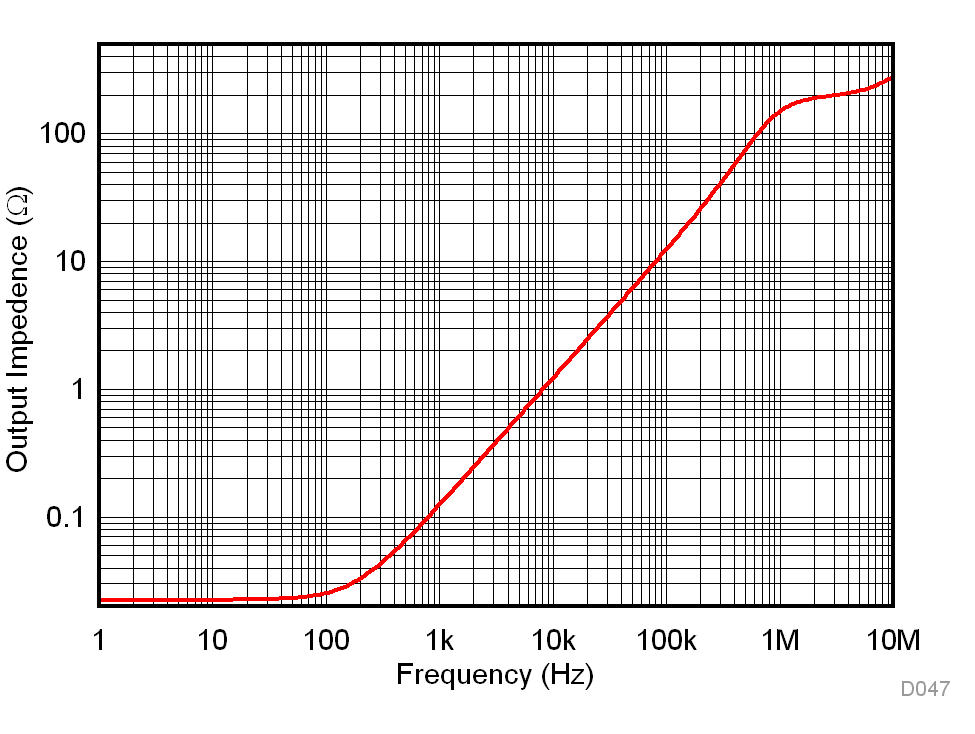Figure 48. Closed-Loop Output Impedance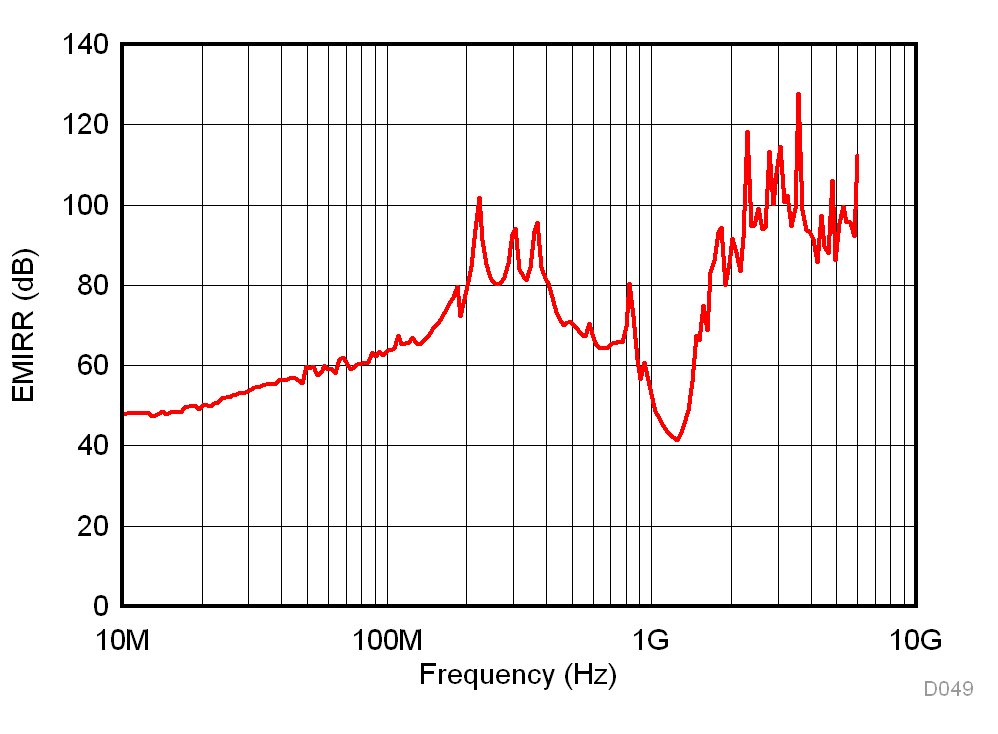Figure 50. Common-Mode EMI Rejection Ratio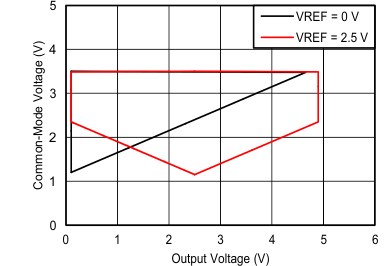VS = 5 V G = 100
Figure 52. Input Common-Mode Voltage vs Output Voltage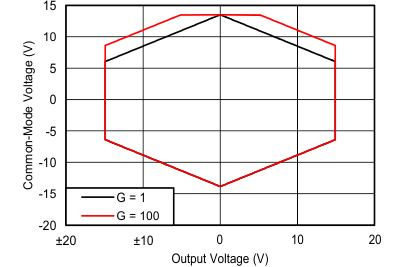VS = ±15 V VREF = 0 V
Figure 54. Input Common-Mode Voltage vs Output Voltage